# Local Spatial Autocorrelation (1)

## Introduction

In this chapter, we will explore the analysis of local spatial autocorrelation statistics, focusing on commonly used univariate measures. We will cover the Local Moran, Local Geary, Getis-Ord statistics, and the more recently developed local join count statistic. We will explore how they can be utilized to discover hot spots and cold spots in the data, as well as spatial outliers. To illustrate these techniques, we will use the Guerry data set on moral statistics in 1830 France, which comes pre-installed with GeoDa.

### Objectives

• Identify clusters with the Local Moran cluster map and significance map

• Identify clusters with the Local Geary cluster map and significance map

• Identify clusters with the Getis-Ord Gi and Gi* statistics

• Identify clusters with the Local Join Count statistic

• Interpret the spatial footprint of spatial clusters

• Assess potential interaction effects by means of conditional cluster maps

• Assess the significance by means of a randomization approach

• Assess the sensitivity of different significance cut-off values

• Interpret significance by means of Bonferroni bounds and the False Discovery Rate (FDR)

#### GeoDa functions covered

• Space > Univariate Local Moran’s I
• significance map and cluster map
• permutation inference
• setting the random seed
• selecting the significance filter
• saving LISA statistics
• select all cores and neighbors
• local conditional map
• Space > Univariate Local Geary
• Space > Local G
• Space > Local G*
• Space > Univariate Join Count

### Getting started

With GeoDa launched and all previous projects closed, we load the Guerry sample data set from the Connect to Data Source interface. As before, we save this file in a working directory, so that we can easily add spatial weights files. We continue to use the ESRI Shape file format, so that we now have a file, say guerry_85 to load. This brings up the familiar themeless base map, showing the 85 French departments, as in Figure 1.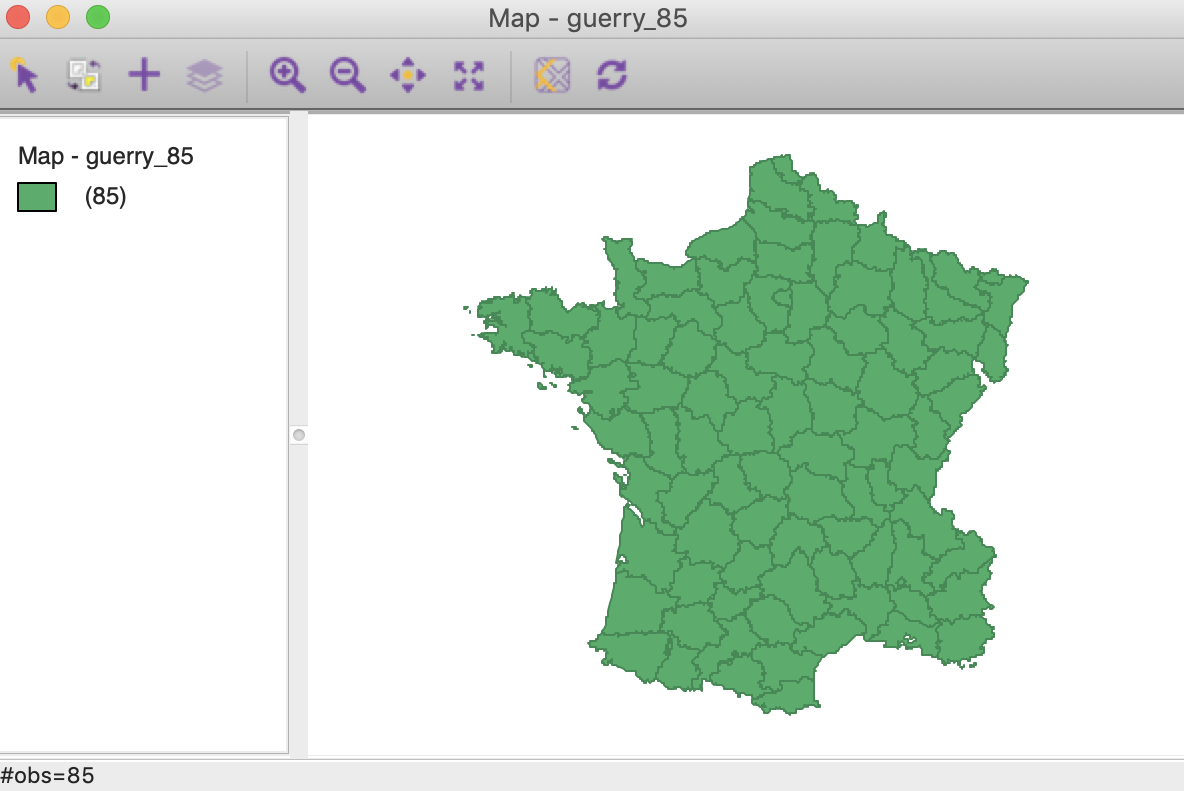Figure 1: French departments themeless map

To carry out the spatial autocorrelation analysis, we will need a spatial weights file, either created from scratch, or loaded from a previous analysis (ideally, contained in a project file). The Weights Manager should have at least one spatial weights file included, e.g., guerry_85_q for first order queen contiguity, as shown in Figure 2.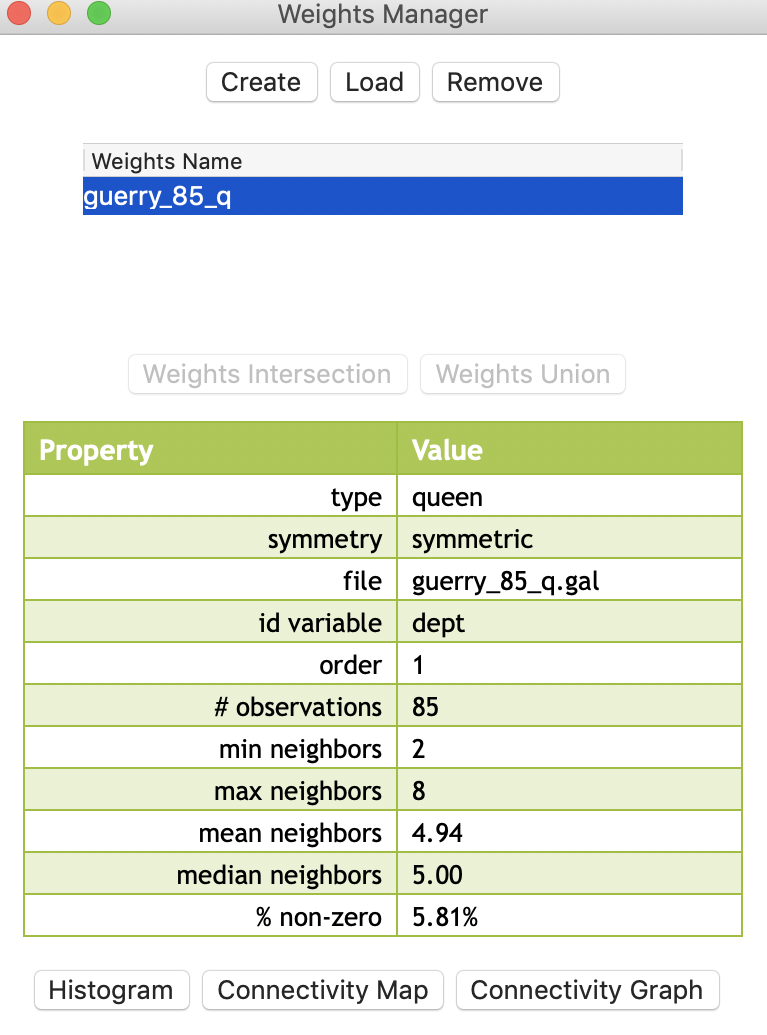Figure 2: Weights manager contents

For this univariate analysis, we will focus on the variable Donatns (charitable donations per capita). This variable displays an interesting spatial distribution, as illustrated in a natural breaks map (using 6 categories), in Figure 3. The global Moran’s I is 0.353, using queen contiguity, and highly significant at p<0.001 (not shown here).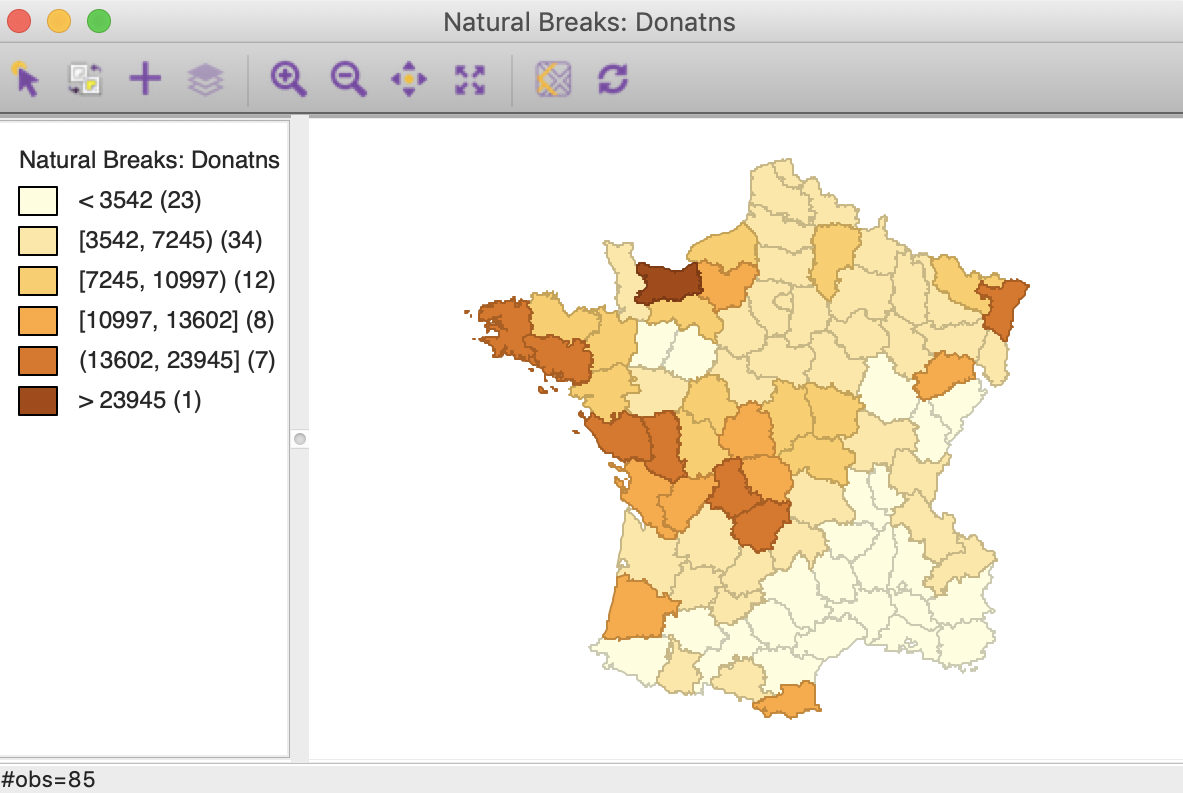Figure 3: Donations – natural breaks map

## Local Moran

### Principle

The Local Moran statistic was suggested in Anselin (1995) as a way to identify local clusters and local spatial outliers. Most global spatial autocorrelation statistics can be expressed as a double sum over the i and j indices, such as $$\sum_i \sum_j g_{ij}$$. The local form of such a statistic would then be, for each observation (location) $$i$$, the sum of the relevant expression over the $$j$$ index, $$\sum_j g_{ij}$$.

Specifically, the Local Moran statistic takes the form $$c. z_i \sum_j w_{ij} z_j$$, with $$z$$ in deviations from the mean. The scalar $$c$$ is the same for all locations and therefore does not play a role in the assessment of significance. The latter is obtained by means of a conditional permutation method, where, in turn, each $$z_i$$ is held fixed, and the remaining z-values are randomly permuted to yield a reference distribution for the statistic. This operates in the same fashion as for the global Moran’s I, except that the permutation is carried out for each observation in turn. The result is a pseudo p-value for each location, which can then be used to assess significance. Note that this notion of significance is not the standard one, and should not be interpreted that way (see the discussion of multiple comparisons below).

Assessing significance in and of itself is not that useful for the Local Moran. However, when an indication of significance is combined with the location of each observation in the Moran Scatterplot, a very powerful interpretation becomes possible. The combined information allows for a classification of the significant locations as high-high and low-low spatial clusters, and high-low and low-high spatial outliers. It is important to keep in mind that the reference to high and low is relative to the mean of the variable, and should not be interpreted in an absolute sense.

### Implementation

The Univariate Local Moran’s I is started from the Cluster Maps toolbar icon, shown in Figure 4.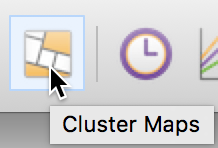Figure 4: Cluster map toolbar icon

It is included as the top level option in the resulting drop down list in Figure 5.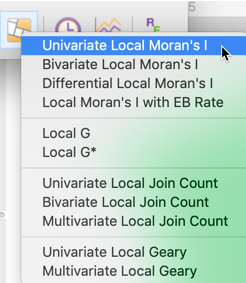Figure 5: Univariate Local Moran from the toolbar

Alternatively, this option can be selected from the main menu, as Space > Univariate Local Moran’s I.

Either approach brings up the familiar Variable Settings dialog in Figure 6, which lists the available variables as well as the default weights file, at the bottom (guerry_85_q). We select Donatns as the variable name.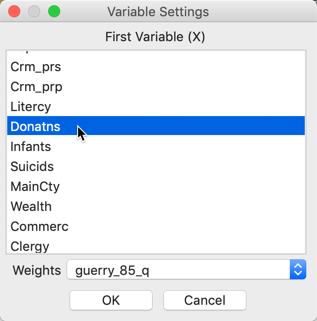Figure 6: Univariate Local Moran variable settings

Clicking OK brings up a dialog to select the number and types of graphs to be created. The default is to provide the Cluster Map only, which is typically the most informative. This is shown in Figure 7. However, in addition, a Significance Map and a Moran Scatter Plot can be brought up as well. To continue, we select the Significance Map as well.Figure 7: Windows options

The results, for the default setting of 999 permutations and a p-value of 0.05 (and with both significance map and cluster map options checked) are illustrated in Figures 8 and 9.

The significance map shows the locations with a significant local statistic, with the degree of significance reflected in increasingly darker shades of green. The maps starts with p < 0.05 and shows all the categories of significance that are meaningful for the given number of permutations. In our example, since there were 999 permutations, the smallest pseudo p-value is 0.001, with four such locations (the darkest shade of green).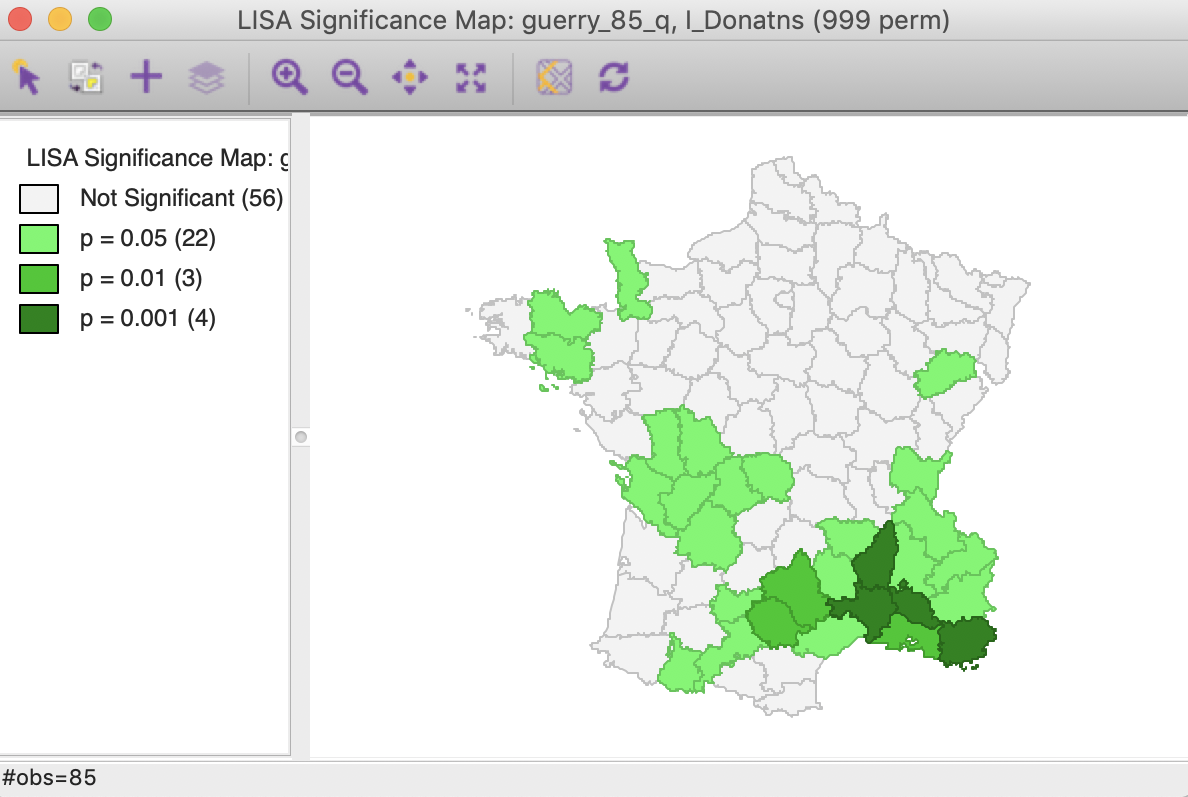Figure 8: Default significance map (p<0.05)

The cluster map augments the significant locations with an indication of the type of spatial association, based on the location of the value and its spatial lag in the Moran scatter plot (see also the discussion below). In this example, all four categories are represented, with dark red for the high-high clusters (eight in our example), dark blue for the low-low clusters (seventeen locations), light blue for the low-high spatial outliers (two locations), and light red for the high-low spatial outliers (two locations).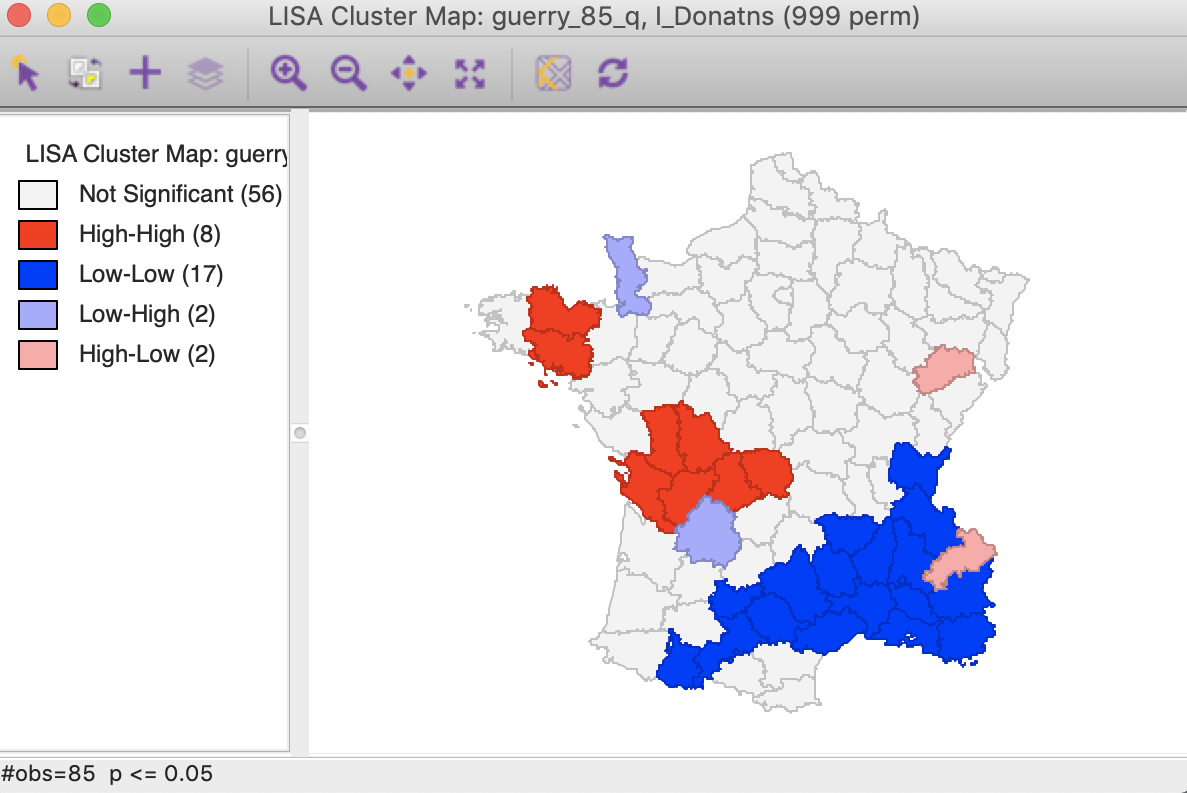Figure 9: Default cluster map (p<0.05)

We now consider the various options and interpretation more closely.

### Randomization options

The Randomization option is the first item in the options menu for both the significance map and the cluster map. It operates in the same fashion as for the Moran scatter plot. As shown in Figure 10, up to 99999 permutations are possible (for each observation in turn), preferably using a specified random seed to allow replication.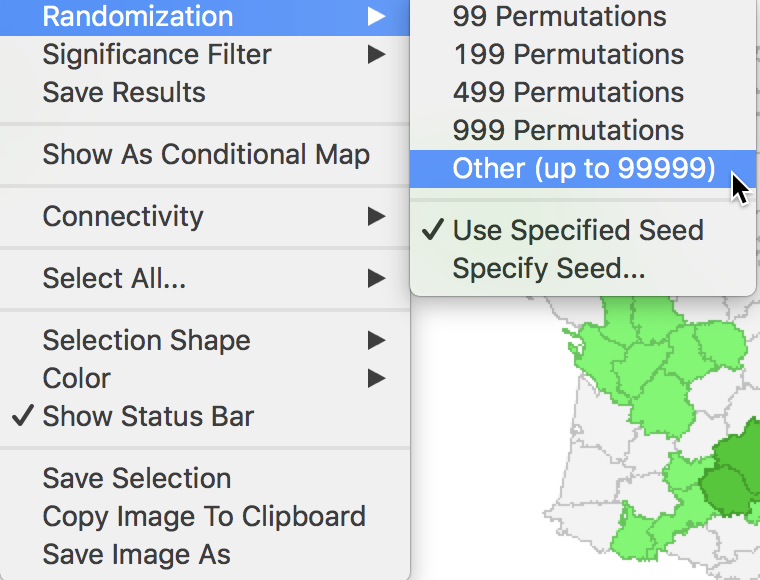Figure 10: Randomization options

The effect of the number of permutations is typically marginal relative to the default of 999. In our example, selecting 99999 results in two minor changes, but the total number of significant locations remains the same. As shown in Figure 11, there are now 21 locations significant at p < 0.05, four at p < 0.01, three at p < 0.001, and one at p < 0.00001, as illustrated by different shades of green in the map.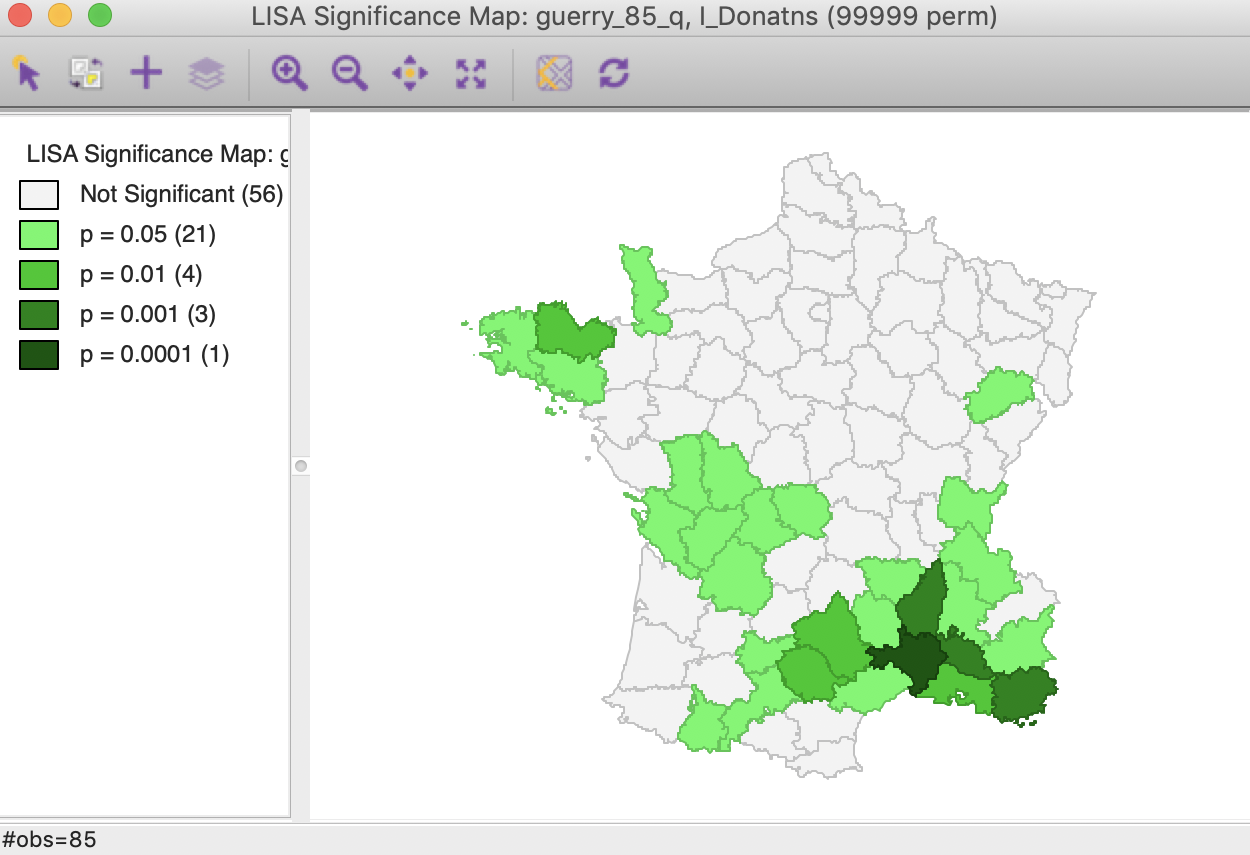Figure 11: Significance map, 99999 permutations

The cluster map is affected in the same two locations, but now we can assess the effect on the four classifications. As illustrated in Figure 12, one of the high-low spatial outliers disappears (adjoining the large low-low cluster in the south of the country, it was significant at p < 0.05 for 999 permutations), and one new high-high cluster is added (to the west of the existing high-high cluster in the Brittany region, also significant at p < 0.05). In general, it is good practice to assess the sensitivity of the significant locations to the number of permutations, although this typically only affects locations with a p-value of 0.05 (see below, for further discussion of the interpretation of significance).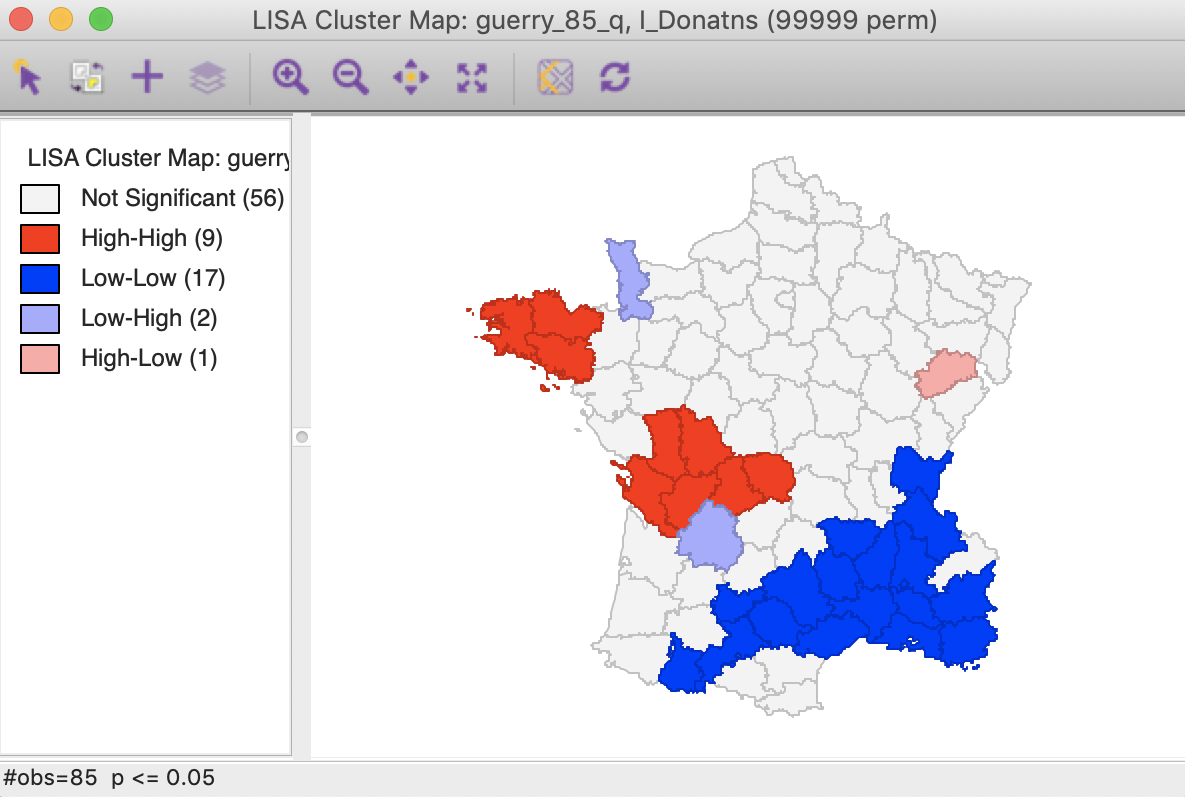Figure 12: Cluster map, 99999 permutations

#### Clusters and outliers

Before moving on to a discussion of significance, we highlight the connection between the Moran scatter plot and the cluster map. As discussed previously, the Moran scatter plot provides a classification of spatial association into four categories, corresponding to the location of the points in the four quadrants of the plot. These categories are referred to as high-high, low-low, low-high and high-low, relative to the mean, which is the center of the graph. It is important to keep in mind that there is a difference between a location (and its spatial lag) being in a given quadrant of the plot, and that location being a significant local cluster or spatial outlier.

To illustrate this point, in the left panel of Figure 13, we select all the locations in the upper right quadrant of the Moran scatter plot. Using the linking feature, they are immediately highlighted in the corresponding cluster map in the right panel of the Figure. The selection is indicated by the red colors in the map, as well as the grey areas that match locations in the plot that are not significant in the map. Whereas there were 22 points selected in the scatter plot, there were only nine locations on the map that were significant (at p < 0.05).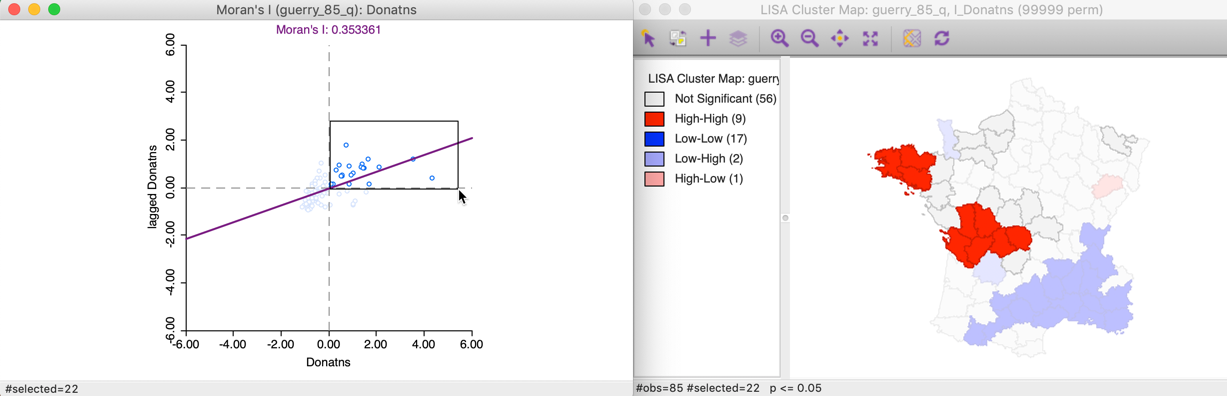Figure 13: High-high Moran scatter plot locations

This can also be illustrated using the reverse logic, starting in the cluster map, by selecting those locations identified as significant high-high cluster centers. In the right hand panel of Figure 14, this is accomplished by clicking on the red rectangle in the legend, next to High-High. All the corresponding cluster centers are shown in red on the map, whereas the other locations are more transparent. Through linking, we can identify the matching nine points in the Moran scatter plot in the left hand panel.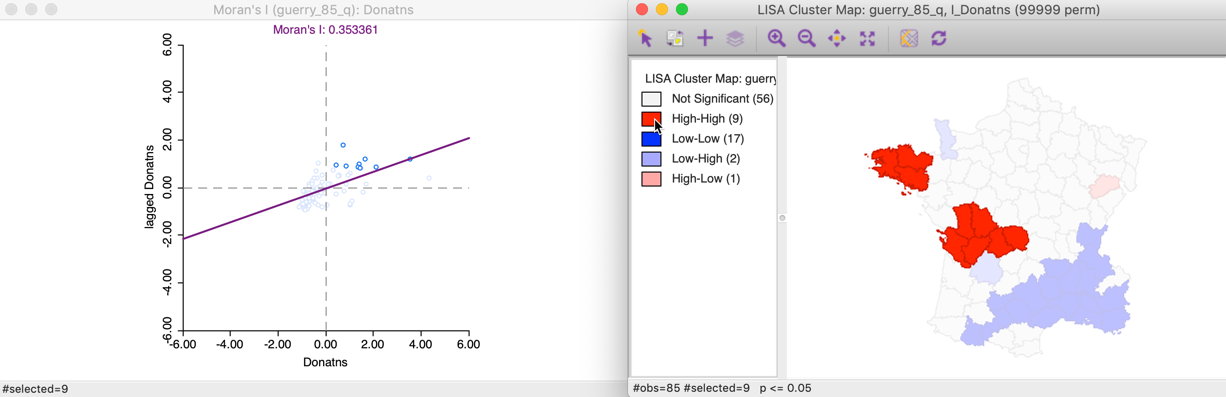Figure 14: High-high cluster locations

Similarly, selecting the Low-High outliers in the cluster map, highlights the corresponding two points in the upper left quadrant of the Moran scatter plot, as shown in Figure 15.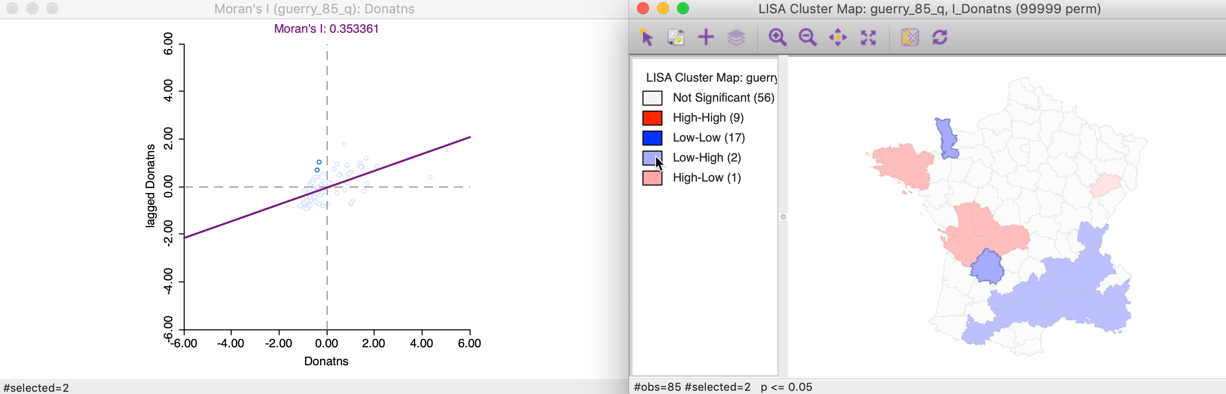Figure 15: Low-high spatial outliers

#### Saving the Local Moran statistics

The Local Moran feature has the typical option to Save Results, selected as the third item in the options menu, shown in Figure 16.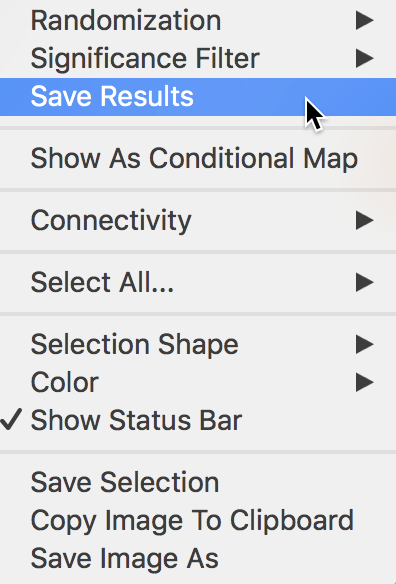Figure 16: Save results option

This brings up a dialog with three potential variables to save to the table, as in Figure 17. The Lisa Indices are the actual values for the local statistics, which are typically not that useful. The next two items are the Clusters and the Significance, i.e., the pseudo p-value.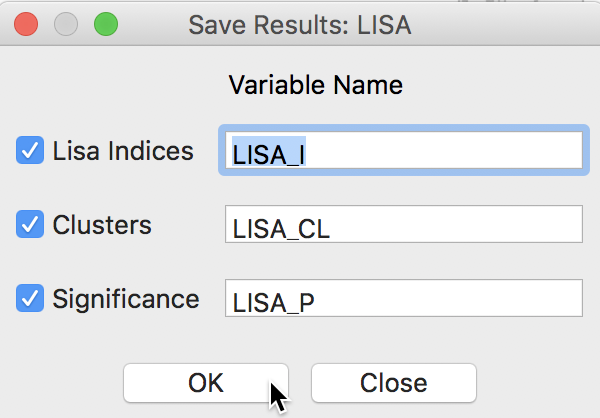Figure 17: LISA variables options

The clusters are identified by an integer that designates the type of spatial association: 0 for non-significant (for the current selection of the p-value, i.e., 0.05 in our example), 1 for high-high, 2 for low-low, 3 for low-high, and 4 for high-low.

Finally, the significance is the pseudo p-value computed from the random permutations.

As before, default variable names are suggested. These would typically be changed, especially when more than one variable is considered (or different spatial weights for the same variable).

Clicking OK adds the variables to the table, as shown in Figure 18. The addition must be made permanent by means of a Save command.Figure 18: LISA variables in table

### Significance

An important methodological issue associated with the local spatial autocorrelation statistics is the selection of the p-value cut-off to properly reflect the desired Type I error. Not only are the pseudo p-values not analytical, since they are the result of a computational permutation process, but they also suffer from the problem of multiple comparisons (for a detailed discussion, see de Castro and Singer 2006). The bottom line is that a traditional choice of 0.05 is likely to lead to many false positives, i.e., rejections of the null when in fact it holds.

There is no completely satisfactory solution to this problem, but GeoDa offers a number of strategies through the Significance Filter item in the options menu, shown in Figure 19.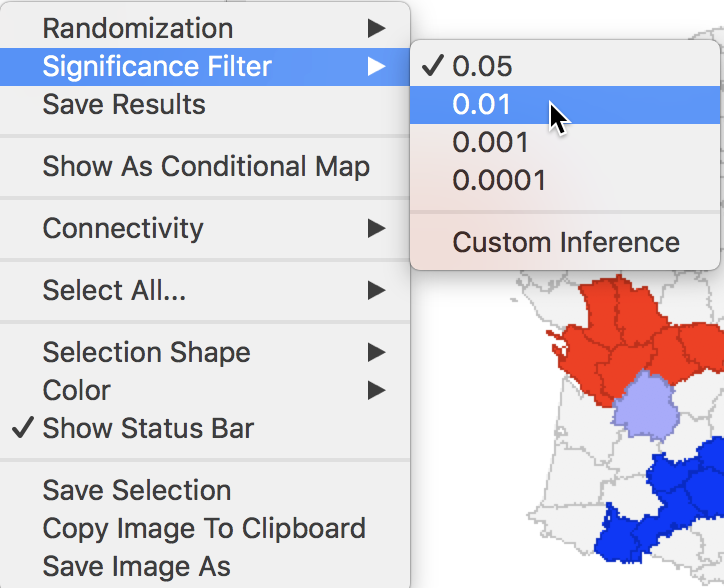Figure 19: Significance filter

A straightforward option is to select one of the pre-selected p-values from the list provided (i.e., 0.05, 0.01, 0.001, or 0.0001). For example, choosing 0.01 immediately changes the locations that are displayed in the significance and cluster maps, as shown in Figure 20. Now, there are only eight significant locations, compared to 29 with a pseudo p-value cut-off of 0.05.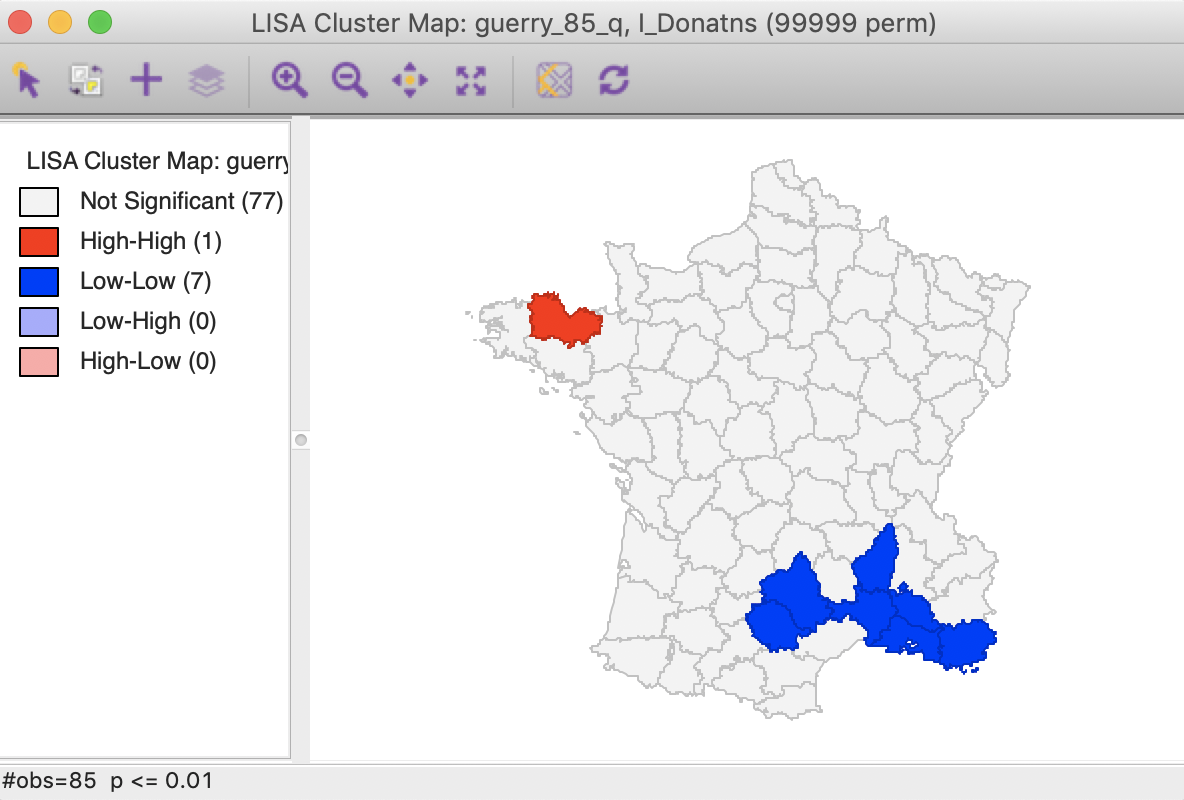Figure 20: Cluster map (p<0.01)

Note that we can obtain exactly the same locations by selecting the three categories of p-values 0.01, 0.001 and 0.0001 in the original significance map of Figure 11 (click on the rectangle next to p=0.01, followed by a shift click on the rectangles next to p = 0.001 and p = 0.0001). The status bar in Figure 21 confirms that the selection consists of eight values (4 for p = 0.01, 3 for p = 0.001, and 1 for p = 0.0001).Figure 21: Selected locations in significance map for p<0.01

A more refined approach to select a proper p-value is available through the Custom Inference item of the significance filter, shown in Figure 22. The associated interface provides a number of options to deal with the multiple comparison problem.Figure 22: Custom inference options

The point of departure is to set a target $$\alpha$$ value for an overall Type I error rate. In a multiple comparison context, this is sometimes referred to as the Family Wide Error Rate (FWER). The target rate is selected in the input box next to Input significance. Without any other options, this is the cut-off p-value used to select the observations. For example, this could be set to 0.1, a value suggested by Efron and Hastie (2016) for big data analysis. In our example, since the 85 observations hardly constitute big data, we keep the value at 0.01.

We now consider the two more refined options, i.e., the Bonferroni bound and the False Discovery Rate.

#### Bonferroni bound

The first custom option in the inference settings dialog is the Bonferroni bound procedure. This constructs a bound on the overall p-value by taking $$\alpha$$ and dividing it by the number of multiple comparisons. In our context, the latter corresponds to the number of observations, $$n$$. As a result, Bonferroni bound would be $$\alpha / n = 0.00012$$, the cut-off p-value to be used to determine significance.

Note that in their recent overview of computer age statistical inference, Efron and Hastie (2016) suggest the use of the term interesting observations, rather than signficant, which we will adopt as well.

Checking the Bonferroni bound radio button in the dialog updates the significance and cluster maps. Only one observation meets this criterion in the sense that its pseudo p-value is less than the cut off, which is confirmed by the cluster map shown in Figure 23.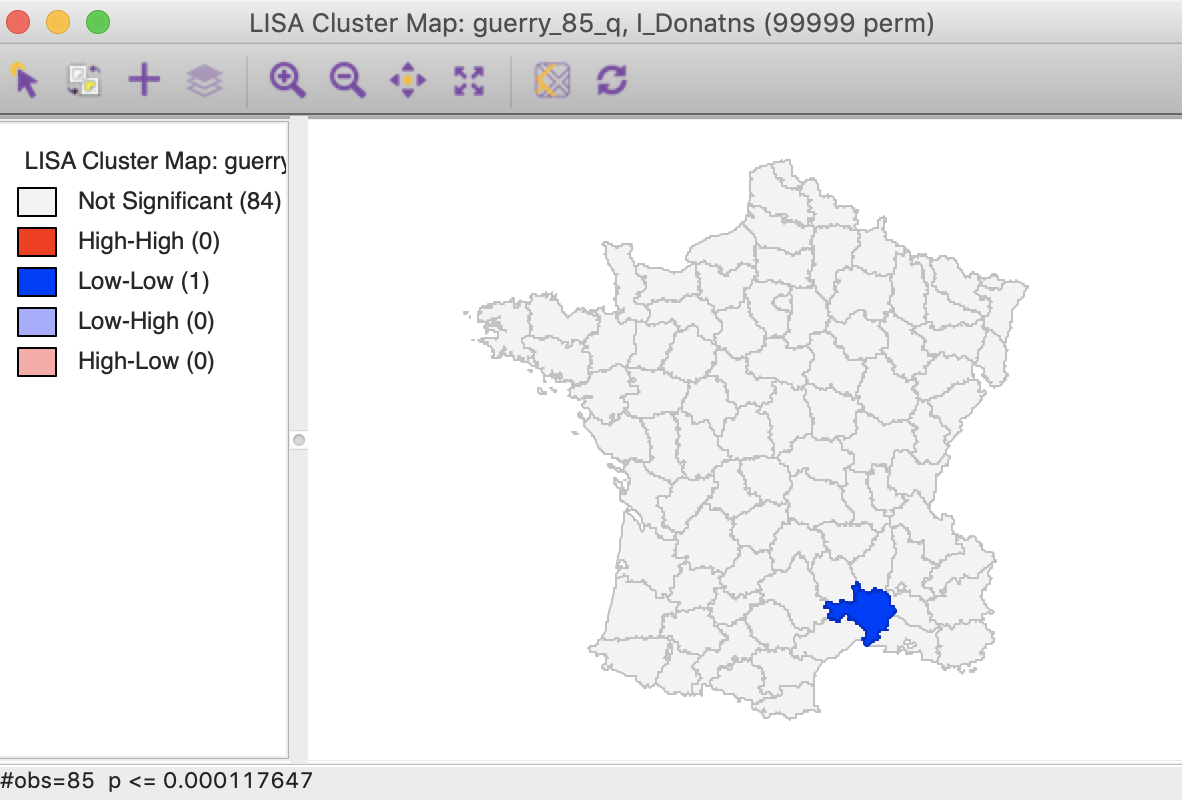Figure 23: Cluster map, Bonferroni (p<0.00012)

#### False Discovery Rate (FDR)

A slightly less conservative option is to use the False Discovery Rate (FDR), first proposed by Benjamini and Hochberg (1995). To illustrate this method, we add some columns to the data table. First, we sort the p-values in increasing order, and add a variable to reflect that order, e.g., the variable I shown in Figure 24, computed in the Table Calculator as Special > Enumerate.

Next, we create a new variable (FDR) that equals $$i \times \alpha / n$$, where $$i$$ is the sequence number of the sorted observations (not the original observation order), $$\alpha$$ is the target, and $$n$$ is the number of observations.2 In our example, $$\alpha$$ is 0.01 and $$n$$ is 85, so that $$1 \times 0.01 / 85 = 0.000118$$ is the first entry. The second entry is $$2 \times 0.01 / 85 = 0.000235$$, etc., as illustrated in the FDR column in the table shown in Figure 24.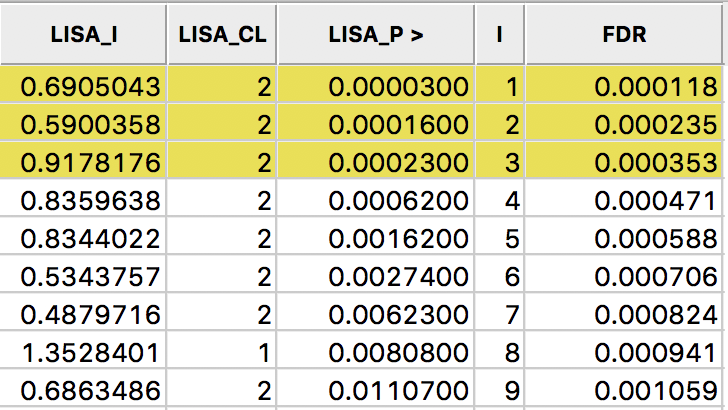Figure 24: Sorted pseudo p-values

We now determine the p-value in the sorted list that corresponds with the sequence number $$i_{max}$$, the largest value for which $$p_{i_{max}} \leq i \times \alpha / n$$. In the table with the sorted p-values, we can see how the first three observations meet the criterion, but for the fourth observation, the pseudo p-value is larger than the value in the FDR column. Consequently, this criterion identifies three locations as significant. Checking the False Discovery Rate radio button will update the signficance and cluster maps accordingly, displaying only three significant locations, as shown in Figure 25.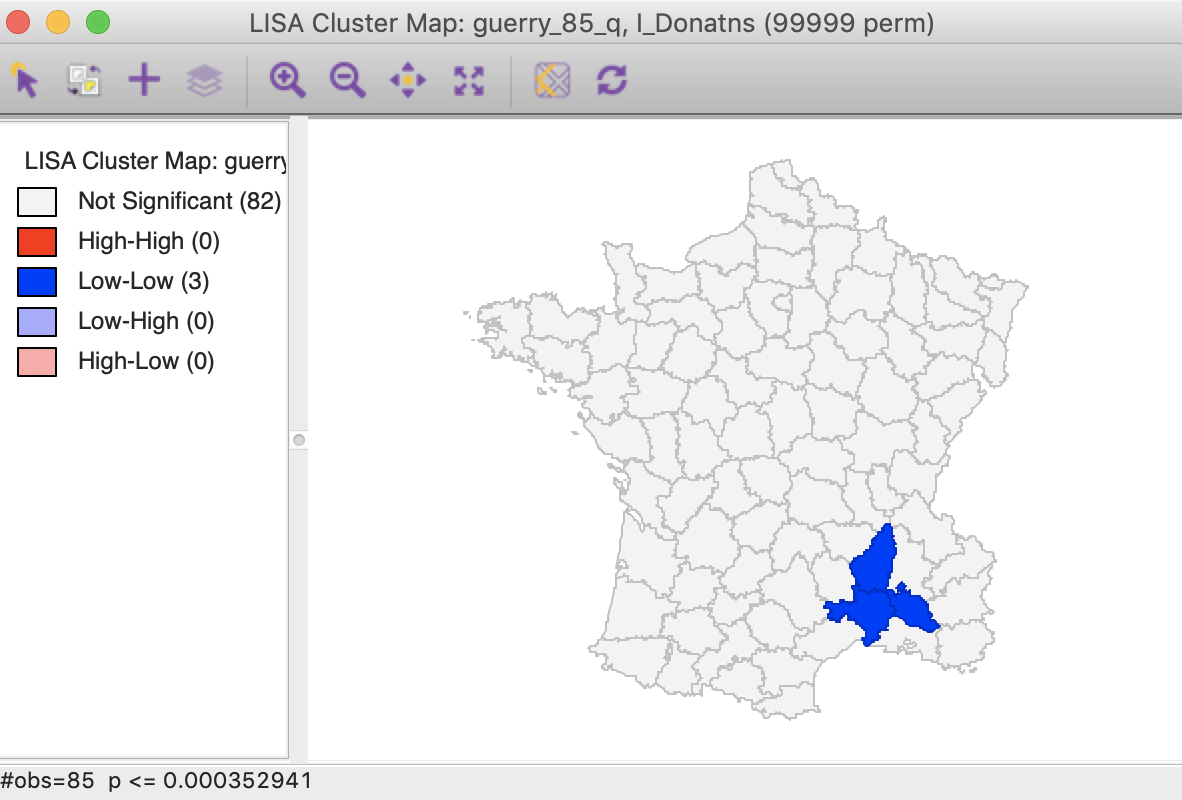Figure 25: Cluster map, FDR (p<0.00035)

#### Interpretation of significance

As mentioned, there is no fully satisfactory solution to deal with the multiple comparison problem. Therefore, it is recommended to carry out a sensitivity analysis and to identify the stage where the results become interesting. A mechanical use of 0.05 as a cut off value is definitely not the proper way to proceed.

Also, for the Bonferroni and FDR procedures to work properly, it is necessary to have a large number of permutations, to ensure that the minimum p-value can be less than $$\alpha / n$$. Currently, the largest number of permutations that GeoDa supports is 99999, so that in order to be meaningful, we must have at least $$\alpha / n \gt 0.00001$$. Otherwise, the Bonferroni criterion cannot yield a single significant value. This is not due to a characteristic of the data, but to the lack of sufficient permutations to yield a pseudo p-value that is small enough.

In practice, this means that with $$\alpha = 0.01$$, data sets with $$n > 1000$$ will not have a significant location using the Bonferroni criterion. With $$\alpha = 0.05$$, this value increases to 5000, and with $$\alpha = 0.1$$ to 10,000. However, for truly large data sets, an uncritical application of the Bonferroni bounds will not give meaningful results.

### Interpretation of clusters

Strictly speaking, the locations shown as significant on the significance and cluster maps are not the actual clusters, but the cores of a cluster. In contrast, in the case of spatial outliers, they are the actual locations of interest.

In order to get a better sense of the spatial extent of the cluster, there are a number of ways to highlight cores, their neighbors, or both in the Select All… option in Figure 26.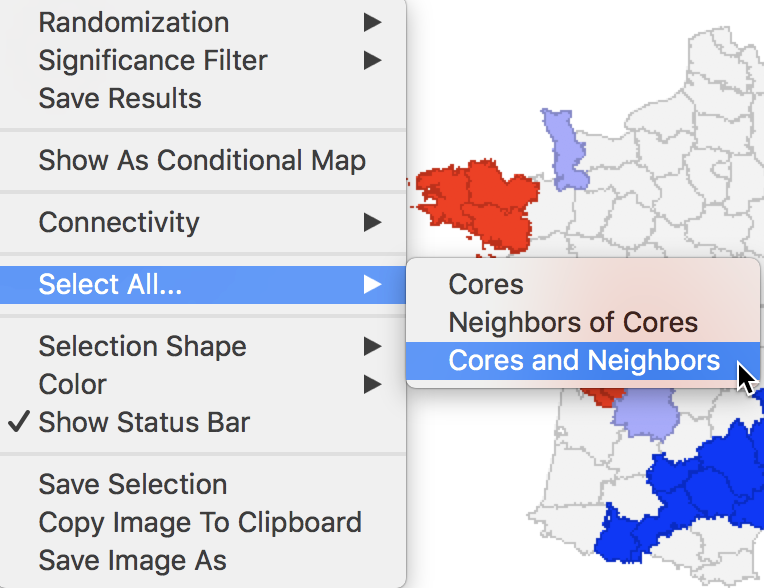Figure 26: Cores and neighbors option

The first option selects the cores, i.e., all the locations shown as non-white in the map. This is not so much relevant for the cluster or significance map, but rather for any maps that are linked. The selection of the Cores will select the corresponding observations in any other map or graph window.

The next option does not select the cores themselves, but their neighbors. Again, this is most relevant when used in combination with linked maps or graphs.

The third option selects both the cores and their neighbors (as defined by the spatial weights). This is most useful to assess the spatial range of the areas identified as clusters. For example, with the p-value set at 0.01, selection of the cores and neighbors yields the regions in Figure 27, with the non-significant neighbors shown in grey.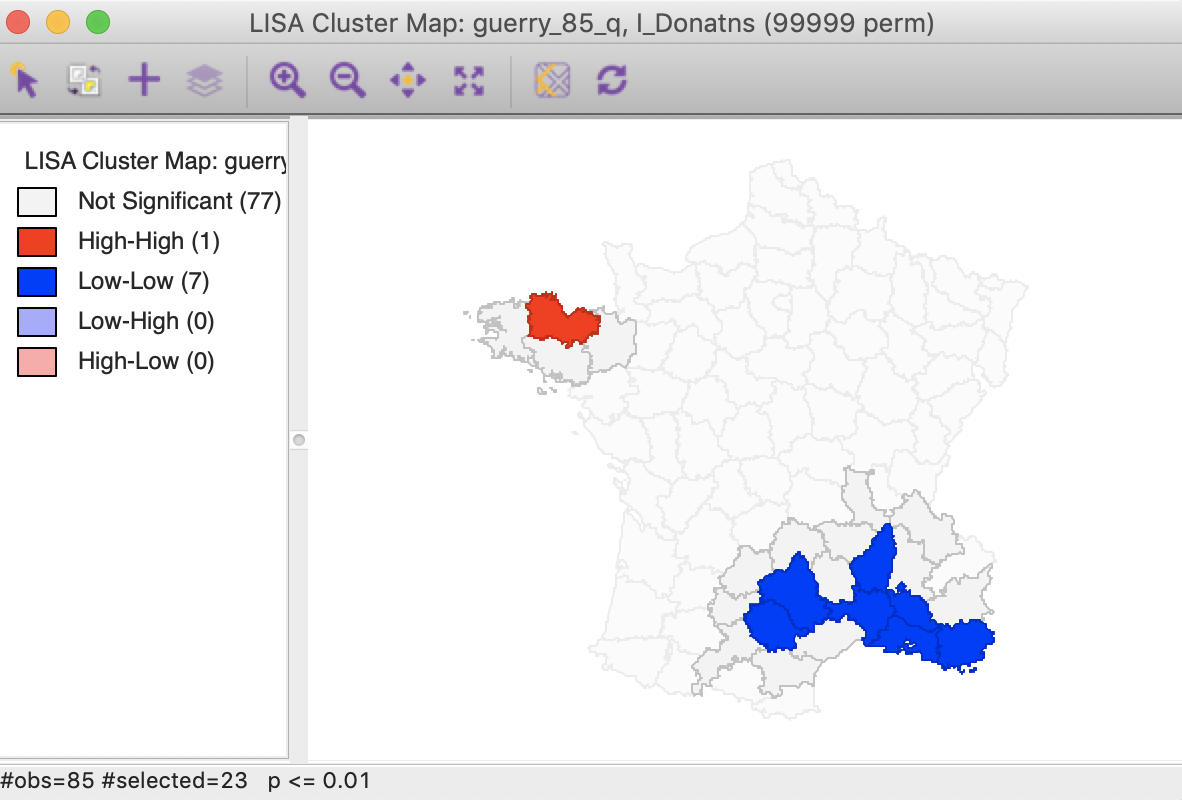Figure 27: Cluster cores and neighbors (p<0.01)

An interesting application of this feature is to superimpose the cores and neighbors selected for a given p-value, say 0.01, onto the cluster cores identified with a different p-value, say 0.05. In the example in Figure 28, the cores and clusters for 0.01 mostly match the locations identified as cluster cores at p < 0.05. In our example, there are 23 locations selected, compared to 26 significant high-high and low-low locations for p < 0.05. The major difference is that the high-high region in the center of the country is totally missed, but the high-high cluster in Brittany and the low-low cluster in the south of the country is almost exactly matched.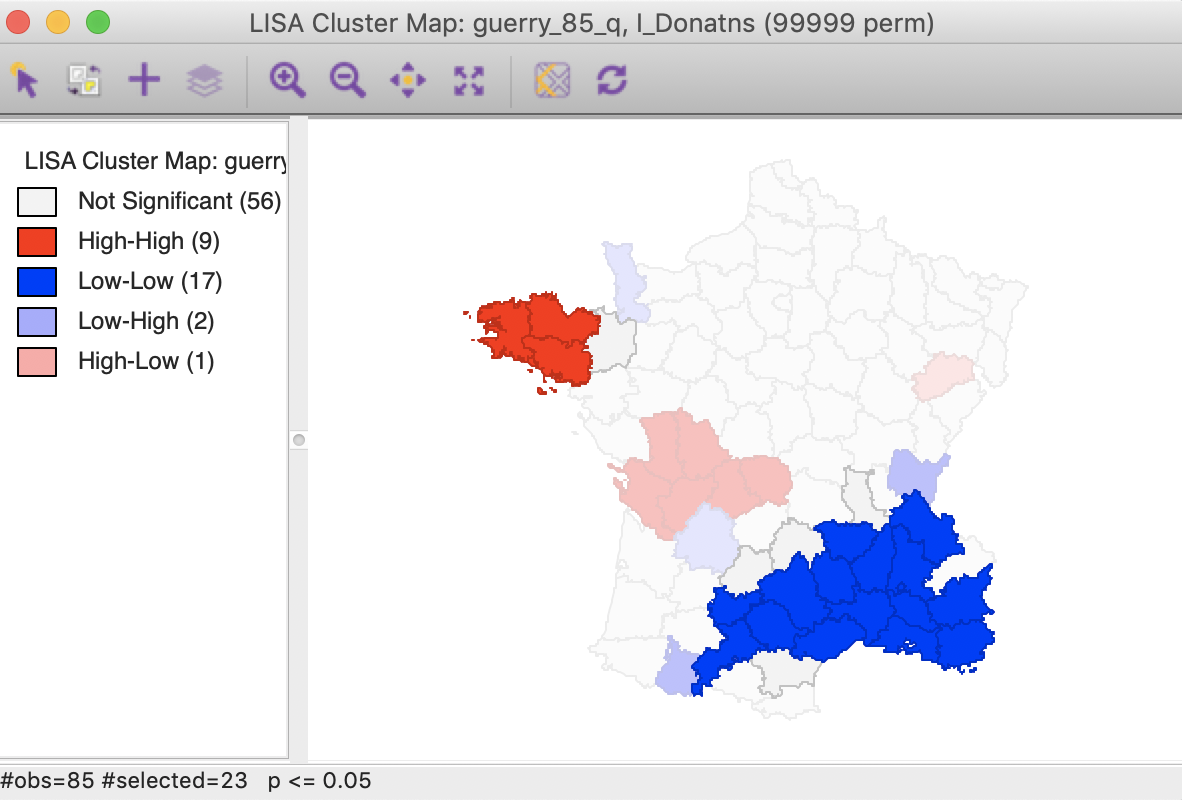Figure 28: Cluster cores and neighbors for p<0.01 overlaid on cluster map for p<0.05

Similarly, checking the cores and neighbors selected in the significance map, clearly illustrates how some of the neighbors at p < 0.01 are identified as significant cores for p < 0.05, as shown in Figure 29.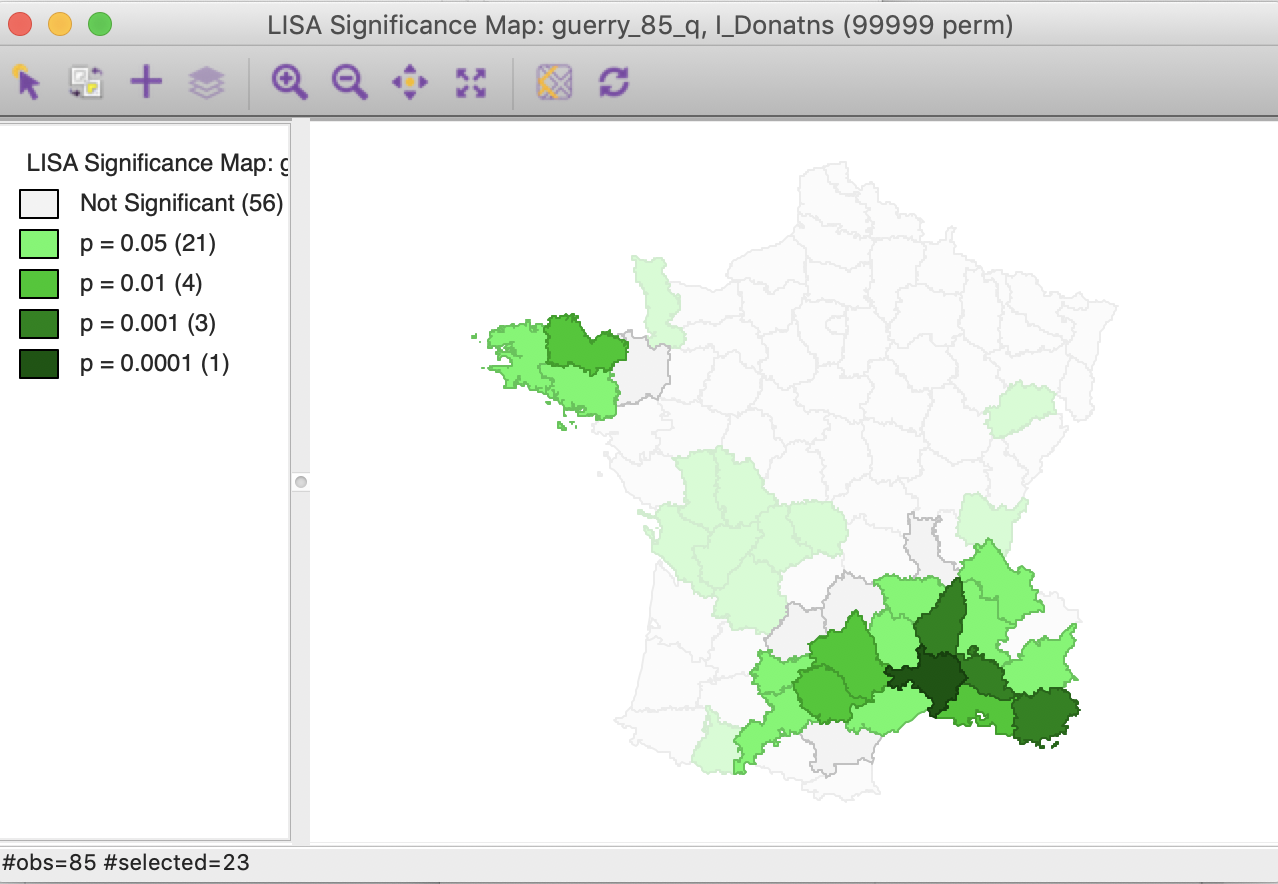Figure 29: Cluster cores and neighbors for p<0.01 in significance map

In sum, this drives home the message that a mechanical application of p-values is to be avoided. Instead, a careful sensitivity analysis should be carried out, comparing cores of clusters identified for different p-values, including the Bonferroni and FDR criteria, as well as the associated neighbors to suggest interesting locations that may suggest new hypotheses or discover the unexpected.

### Conditional local cluster maps

A final option for the Local Moran statistic is that the cluster maps can be incorporated in a conditional map view, similar to the conditional maps we covered earlier. This is accomplished by selecting the Show As Conditional Map option in Figure 30.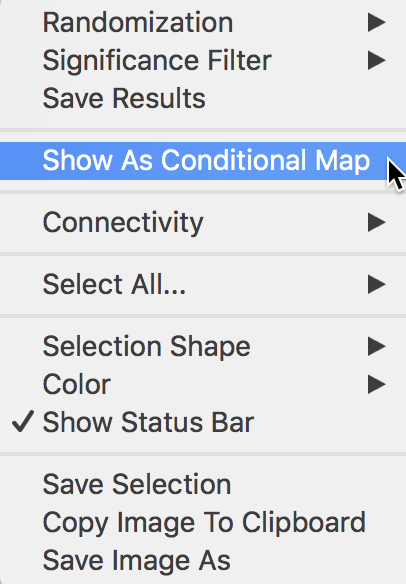Figure 30: Conditional map option

The resulting dialog is the same as for the standard conditional map we reviewed in an earlier chapter. In our example, we take literacy (Litercy) as the conditioning variable for the x-axis, and Clergy as the conditioning variable for the y-axis. In addition, we change the default 3 by 3 micromaps to a 2 by 2 setup, selecting the median as the cut point for each variable (select quantile > 2).

The result is as shown in Figure 31, depicting four micromaps. The maps on the left show the location of clusters and outliers (using p < 0.05 in this example) for those departments with literacy below the median, whereas the maps on the right show the corresponding departments above the median. The main difference seems to be between the maps on the lower end of clergy versus the upper end. The upper end seems to have more high-high cluster cores, with the lower end with more low-low cores.

However, this example is purely illustrative of the functionality available through the conditional cluster map feature, rather than as a substantive interpretation. As always, the main focus is on whether the micromaps suggest different patterns, which would imply an interaction effect with the conditioning variables.Figure 31: Conditional cluster map

## Local Geary

### Principle

The Local Geary statistic, first outlined in Anselin (1995), and further elaborated upon in Anselin (2018), is a Local Indicator of Spatial Association (LISA) that uses a different measure of attribute similarity. As in its global counterpart, the focus is on squared differences, or, rather, dissimilarity. In other words, small values of the statistics suggest positive spatial autocorrelation, whereas large values suggest negative spatial autocorrelation.

Formally, the Local Geary statistic is $LG_i = \sum_j w_{ij}(x_i - x_j)^2,$ in the usual notation.

Inference is again based on a conditional permutation procedure and is interpreted in the same way as for the Local Moran statistic. However, the interpretation of significant locations in terms of the type of association is not as straightforward. In essence, this is because the attribute similarity is not a cross-product and thus has no direct correspondence with the slope in a scatter plot. Nevertheless, we can use the linking capability within GeoDa to make an incomplete classification.

Those locations identified as significant and with the Local Geary statistic smaller than its mean, suggest positive spatial autocorrelation (small differences imply similarity). For those observations that can be classified in the upper-right or lower-left quadrants of a matching Moran scatter plot, we can identify the association as high-high or low-low. However, given that the squared difference can cross the mean, there may be observations for which such a classification is not possible. We will refer to those as other positive spatial autocorrelation.

For negative spatial autocorrelation (large values imply dissimilarity), it is not possible to assess whether the association is between high-low or low-high outliers, since the squaring of the differences removes the sign.

### Implementation

In the same way as for the Local Moran, the Local Geary can be invoked from the Cluster Maps toolbar icon, as Univariate Local Geary in the drop down menu, shown in Figure 32.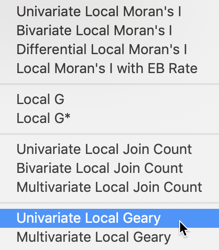Figure 32: Local Geary option from cluster maps toolbar options

Alternatively, it can be started from the main menu, as Space > Univariate Local Geary.

The subsequent step is the same as before, bringing up the Variable Settings dialog that contains the names of the available variables as well as the spatial weights. Everything operates in the same way for all local statistics, so we will not dwell on those aspects here. We again select Donatns as the variable, with guerry_85_q as the queen contiguity weights.

The following dialog offering different window options is slightly different, in that there is no Moran scatter plot option. The only options are for the Significance Map and the Cluster Map. The default is that only the latter is checked, as in Figure 33.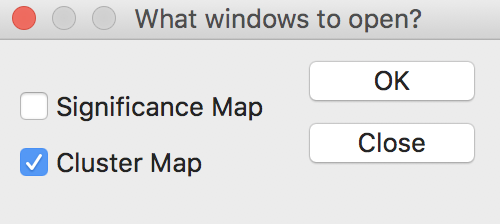Figure 33: Local Geary window options

After selecting the Significance Map option as well, the OK button generates two maps, using a default p-value of 0.05 and 999 permutations, as shown in Figures 34 and 35. In our example, there are 28 significant locations, highlighted in Figure 34.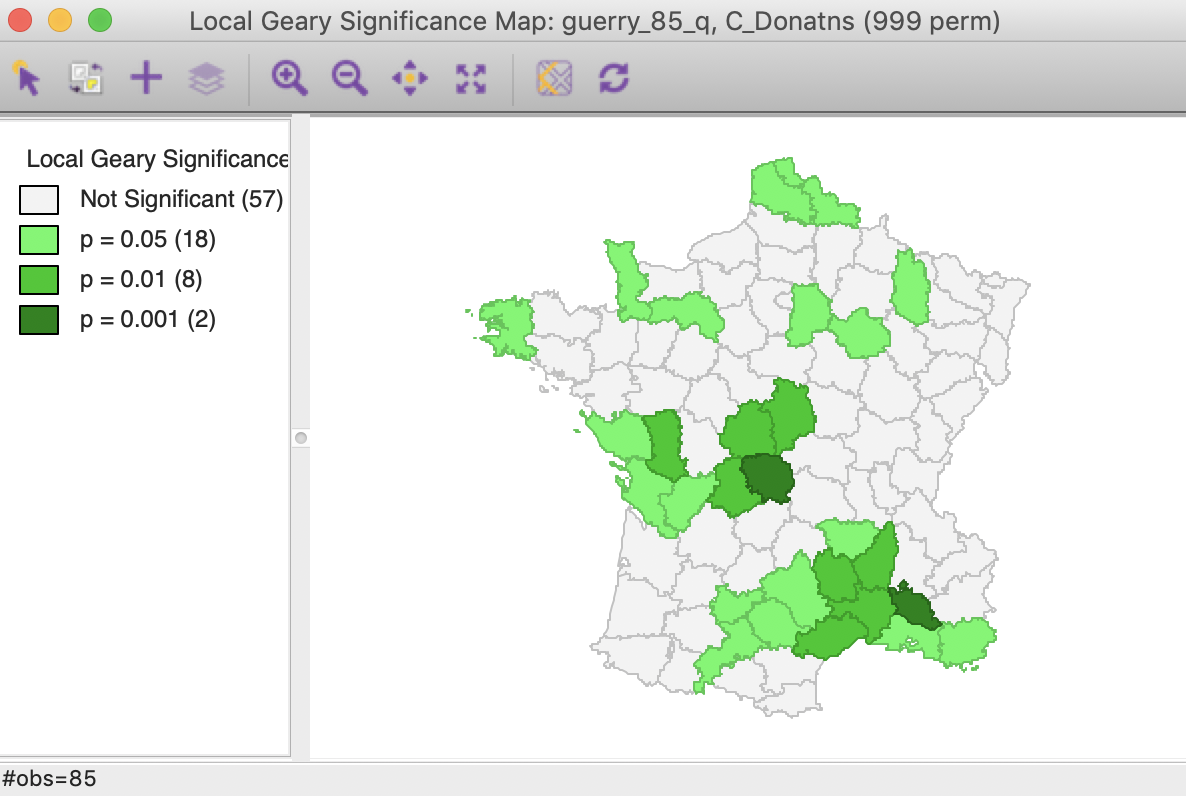Figure 34: Local Geary default significance map (p<0.05)

As discussed above, some of the locations with a positive spatial autocorrelation can be distinguished between the high-high and low-low cases. As shown in Figure 35, there are 9 such high-high locations and 17 low-low locations. There are no locations with positive spatial autocorrelation classified as other in this case. There are two observations with negative spatial autocorrelation, although, as discussed, it is not possible to characterized the type of spatial outliers they correspond with.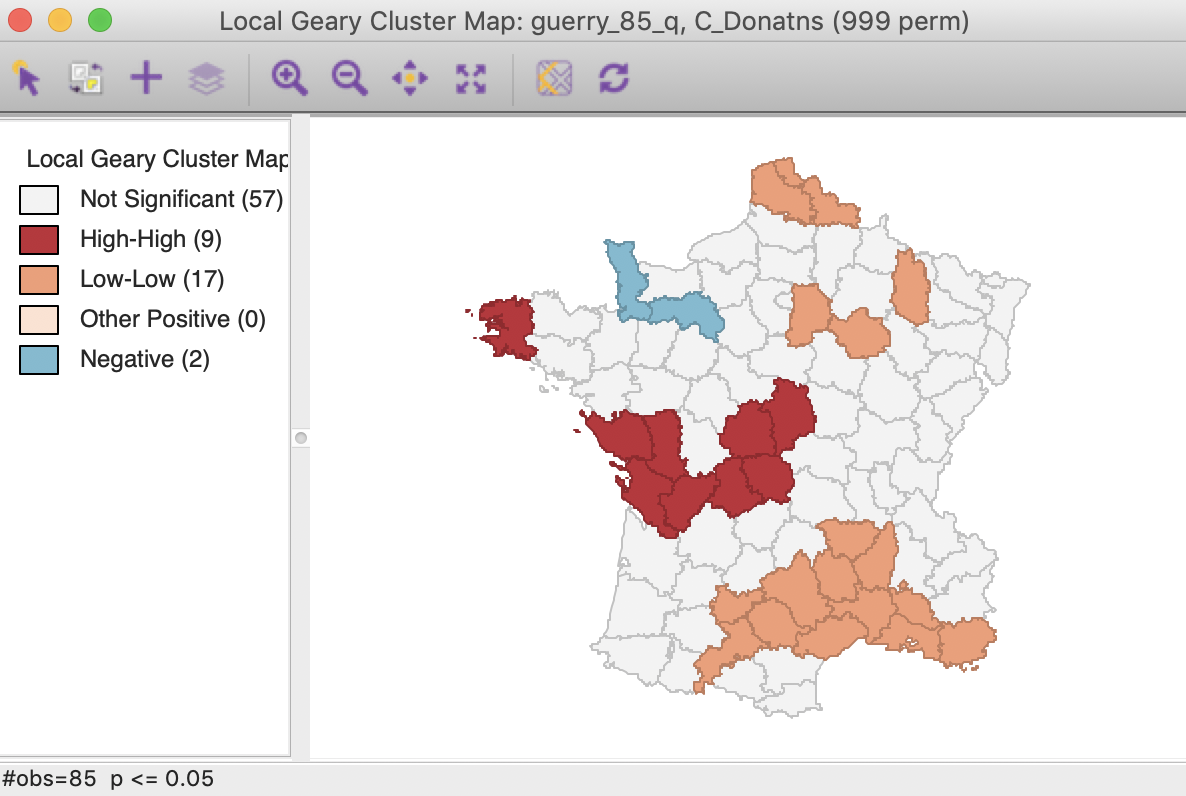Figure 35: Local Geary default cluster map (p<0.05)

All the options operate the same for all local statistics, including the randomization setting, the selection of significance levels, the selection of cores and neighbors, the conditional map option, as well as the standard operations of setting the selection shape and saving the image.

Below, we only discuss the interpretation and how to save the results, which differ slightly in each case.

### Interpretation and significance

Before proceeding further, we change the randomization option to 99999 permutations. This results in minor changes in the cluster map, with two of the marginal (i.e., only significant at p < 0.05) low-low locations removed. As a result, there are now 26 significant locations.

To illustrate the rationale behind the classification of the local clusters, we link the locations identified as high-high with a matching Moran scatter plot. As usual, we select the observations in question by clicking on the red rectangle in the legend next to High-High. This highlights the corresponding locations in the cluster map (the other locations become more transparent) and simultaneously selects the matching points in the Moran scatter plot. As illustrated in Figure 36, the type of association is between locations above the mean and a spatial lag that is also above the mean, which we have characterized as high-high.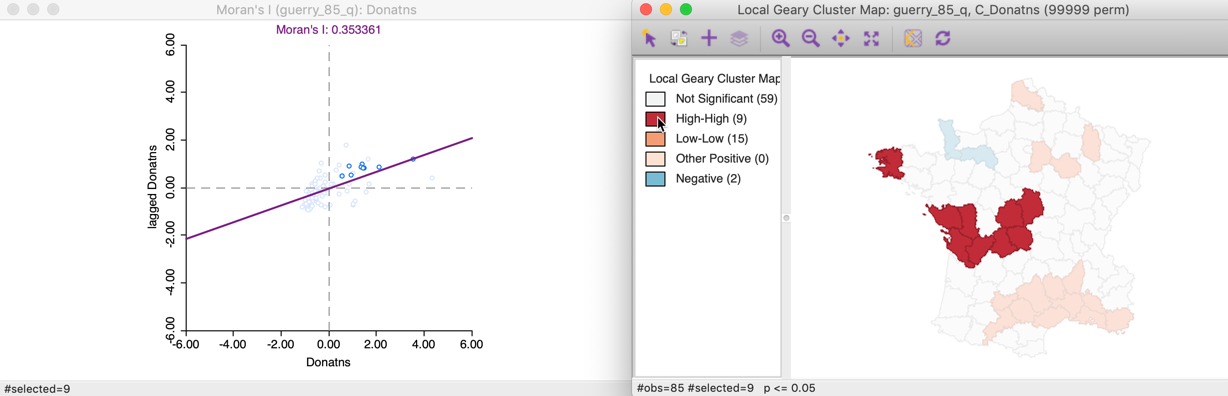Figure 36: Local Geary high-high clusters

Similarly, selecting the low-low cluster cores (click the orange rectangle in the legend) shows the corresponding points in the lower-left quadrant of the Moran scatter plot in Figure 37.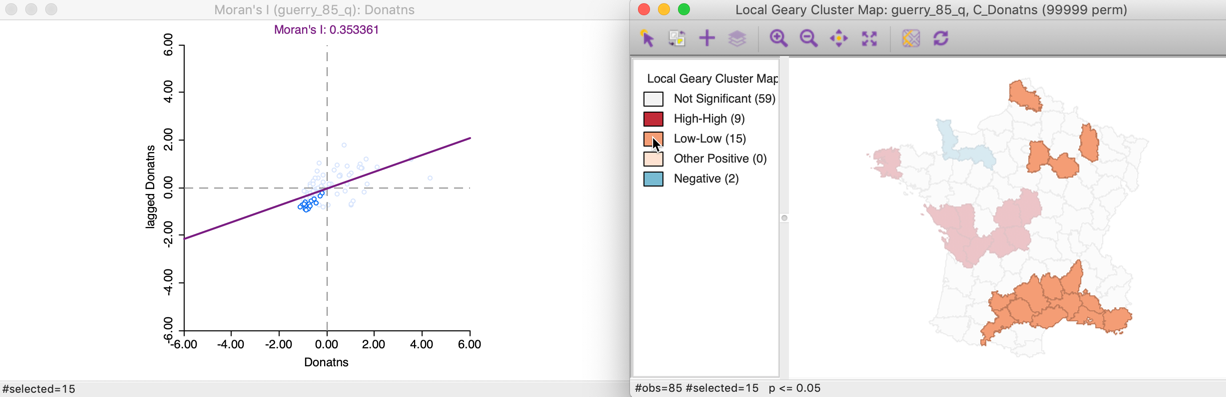Figure 37: Local Geary low-low clusters

For negative spatial autocorrelation, there is no unambiguous classification, since the squared differences eliminate the sign of the dissimilarity between an observation and its neighbors. The corresponding points in the Moran scatter plot are not informative, as shown in Figure 38.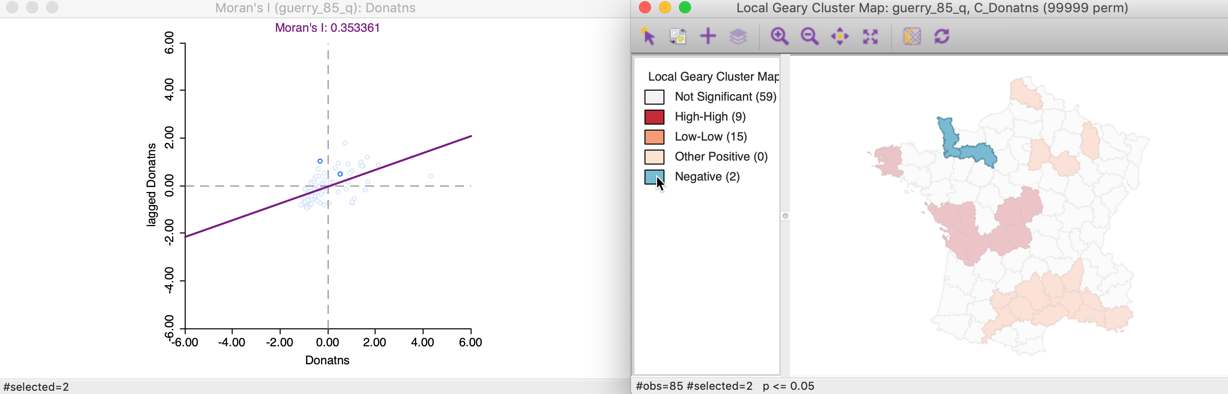Figure 38: Local Geary negative spatial autocorrelation

#### Changing the significance threshold

With 99999 permutations, the significance map allows for a much finer grained assessment of significance. In our example, in Figure 39, 14 locations are significant at 0.05, 10 at 0.01, and one each for 0.001 and 0.00001. Note that there is some correspondence between the Local Moran and the Local Geary cluster maps, but there is by no means a perfect match. Specifically, while the most significant locations are in the same region (the South of France), the location with p < 0.00001 found here is not the same as the one identified for the Local Moran, but a neighbor.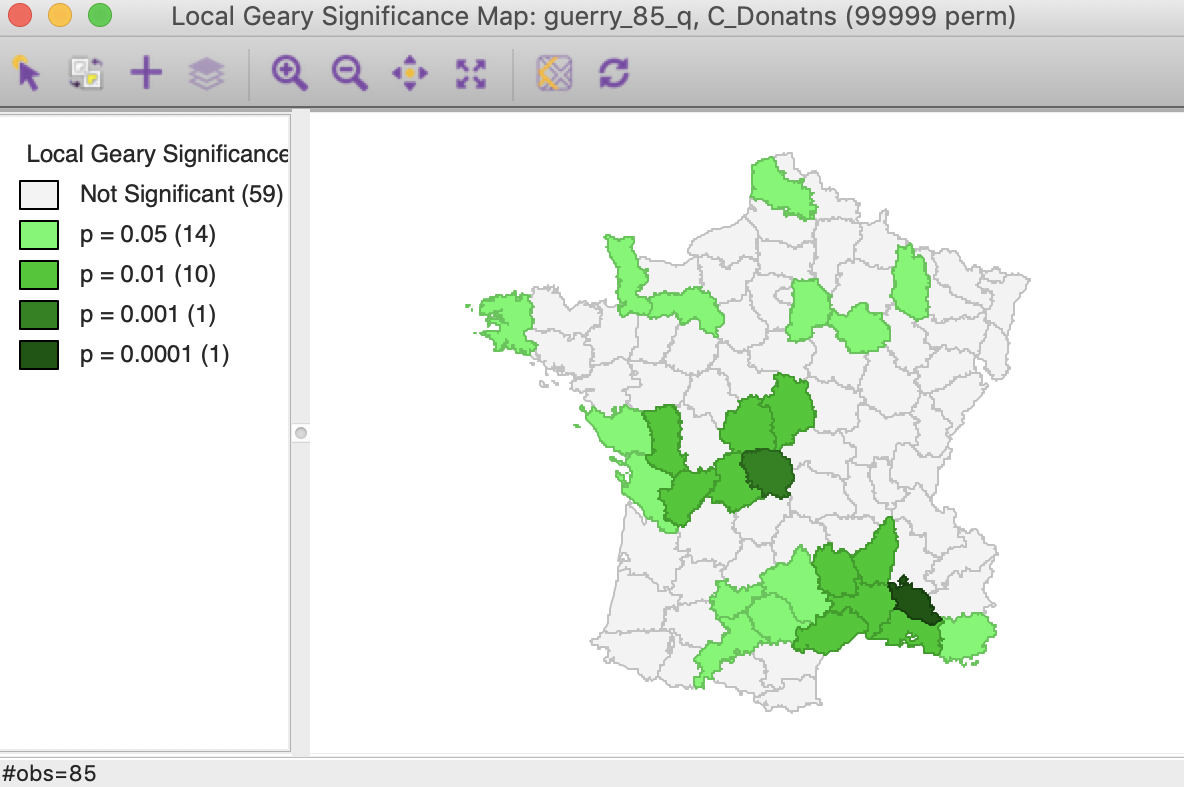Figure 39: Local Geary significance map (99999 permutations)

In the same way as for the Local Moran statistic, we can manipulate the Significance Filter to assess the sensitivity of the identified clusters and spatial outliers to the choice of the cut-off point. For example, in Figure 40, with p < 0.01, there are six high-high and six low-low cluster cores, but there is no longer any evidence of spatial outliers.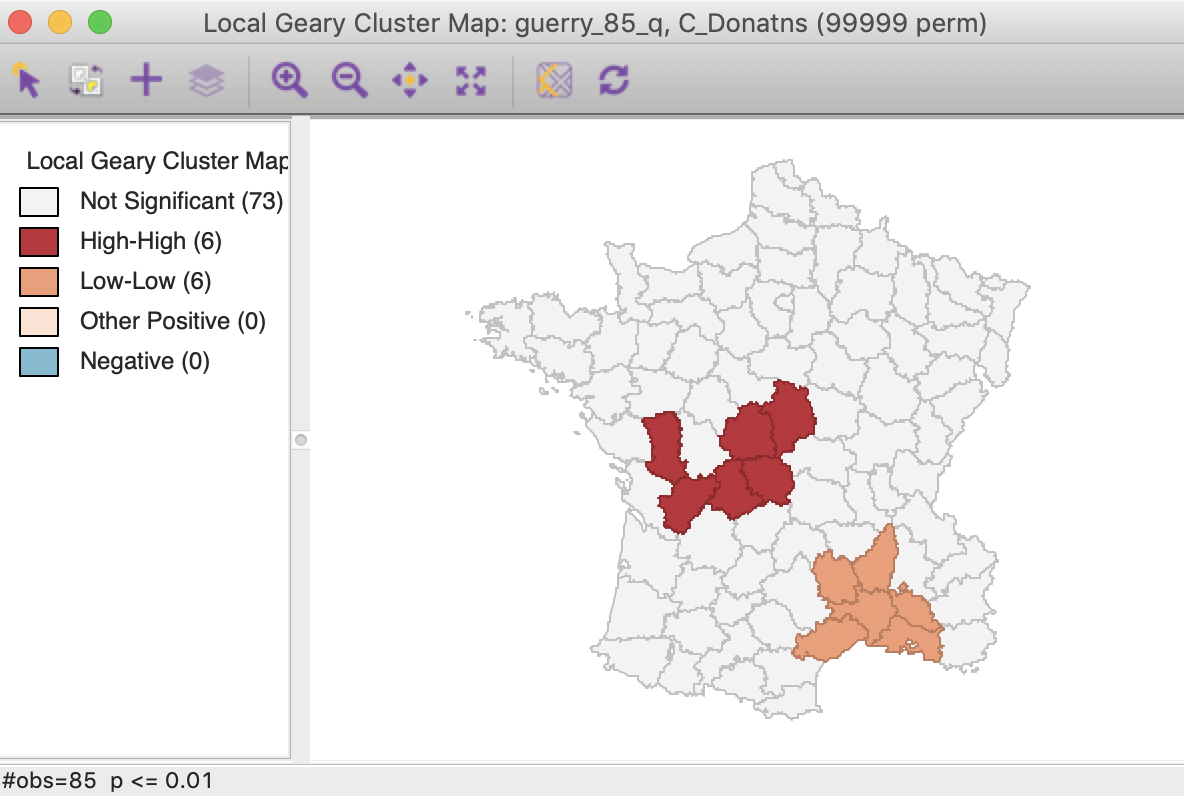Figure 40: Local Geary (p < 0.01)

In this particular case, the Bonferroni bound and the FDR yield the same cut-off value of 0.00012, with only one significant location, highlighted in Figure 41.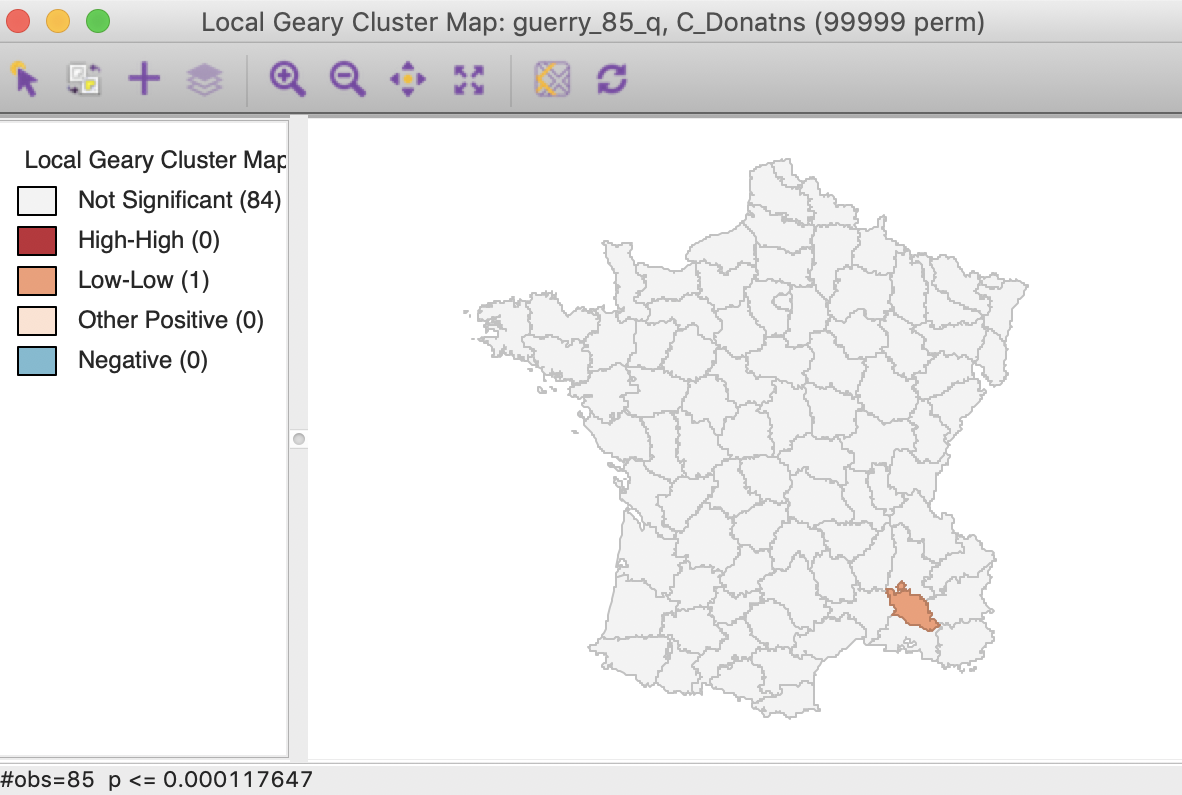Figure 41: Local Geary FDR (p < 0.00012)

#### Saving the results

We can again add selected statistics to the data table by means of the Save Results option. As before, the dialog gives the option to save the statistic itself, the cluster indication and the significance, as shown in Figure 42. Default values for the variable names are suggested, but these will typically need to be customized.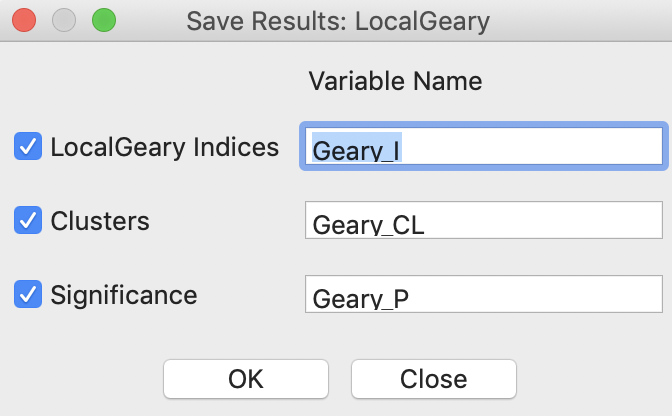Figure 42: Local Geary Save Results options

The code for the cluster classification used for the Local Geary is 0 for not significant, 1 for a high-high cluster core, 2 for a low-low cluster core, 3 for other (positive spatial autocorrelation), and 4 for negative spatial autocorrelation.

As always, any addition to the data table is only made permanent after a Save operation.

## Getis-Ord Statistics

### Principle

A third class of statistics for local spatial autocorrelation was suggested by Getis and Ord (1992), and further elaborated upon in Ord and Getis (1995). It is derived from a point pattern analysis logic. In its earliest formulation the statistic consisted of a ratio of the number of observations within a given range of a point to the total count of points. In a more general form, the statistic is applied to the values at neighboring locations (as defined by the spatial weights). There are two versions of the statistic. They differ in that one takes the value at the given location into account, and the other does not.

The $$G_i$$ statistic consist of a ratio of the weighted average of the values in the neighboring locations, to the sum of all values, not including the value at the location ($$x_i$$). $G_i = \frac{\sum_{j \neq i} w_{ij} x_j}{\sum_{j \neq i} x_j}$

In contrast, the $$G_i^*$$ statistic includes the value $$x_i$$ in both numerator and denominator: $G_i^* = \frac{\sum_j w_{ij} x_j}{\sum_j x_j}.$ Note that in this case, the denominator is constant across all observations and simply consists of the total sum of all values in the data set.

The interpretation of the Getis-Ord statistics is very straightforward: a value larger than the mean (or, a positive value for a standardized z-value) suggests a high-high cluster or hot spot, a value smaller than the mean (or, negative for a z-value) indicates a low-low cluster or cold spot. In contrast to the Local Moran and Local Geary statistics, the Getis-Ord approach does not consider spatial outliers.3

Inference is based on conditional permutation, using an identical procedure as for the other statistics.

### Implementation

The implementation of the Getis-Ord statistics is largely identical to that of the other local statistics. Each statistic an be selected from the drop down menu generated by the Cluster Maps toolbar icon, either as Local G, or as Local G* in Figure 43.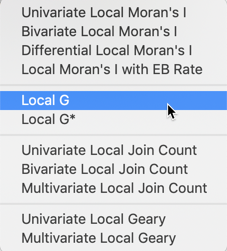Figure 43: Getis-Ord statistics from cluster maps toolbar options

Alternatively, the same two options are also available from the main menu, as Space > Local G or Space > Local G*.

The next step brings up the Variable Settings dialog. Again, we select Donatns as the variable, with guerry_85_q as the queen contiguity weights. This is followed by a choice of windows to be opened. The latter is again slightly different from the previous cases. The default, shown in Figure 44, is to use row-standardized weights and to generate only the Cluster Map. The Significance Map option needs to be invoked explicitly by checking the corresponding box.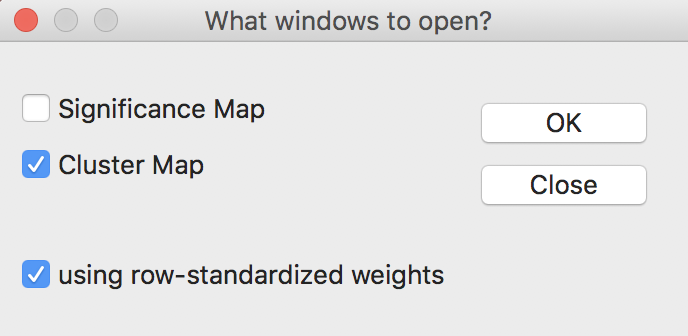Figure 44: Getis-Ord statistics window options

The Getis-Ord statistics also allow the use of binary weights (i.e., not row-standardized), by having the row-standardized weights box unchecked. In practice, the results rarely differ much.

Using the default settings of 999 permutations, with p at 0.05, yields the significance map for the $$G_i$$ statistic shown in Figure 45.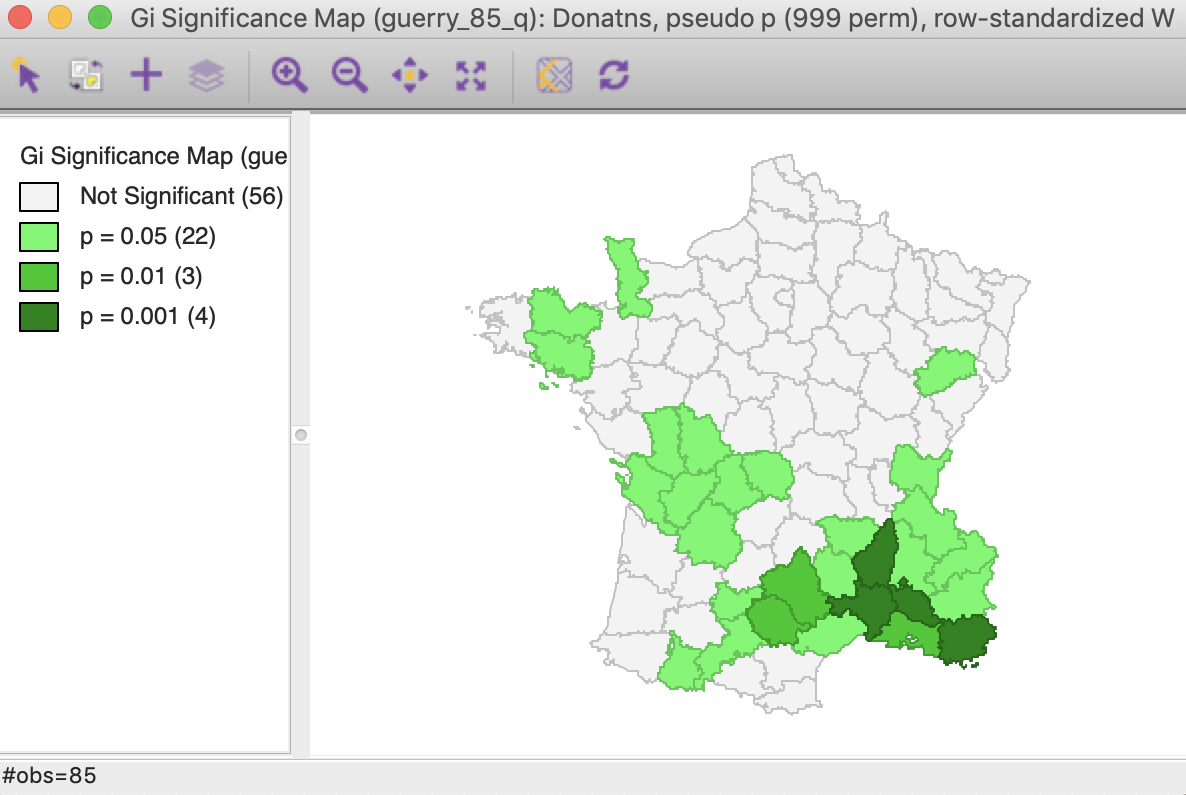Figure 45: Gi statistic default significance map (999 permutations)

The corresponding cluster map, illustrated in Figure 46, shows 10 high-high cluster cores or hot spots (in red on the map), and 19 low-low cluster cores or cold spots (in blue on the map). Note that these are the exact same locations as identified for the Local Moran, except that the spatial outliers are now classified as part of the clusters (one in the high-high group and one in the low-low group).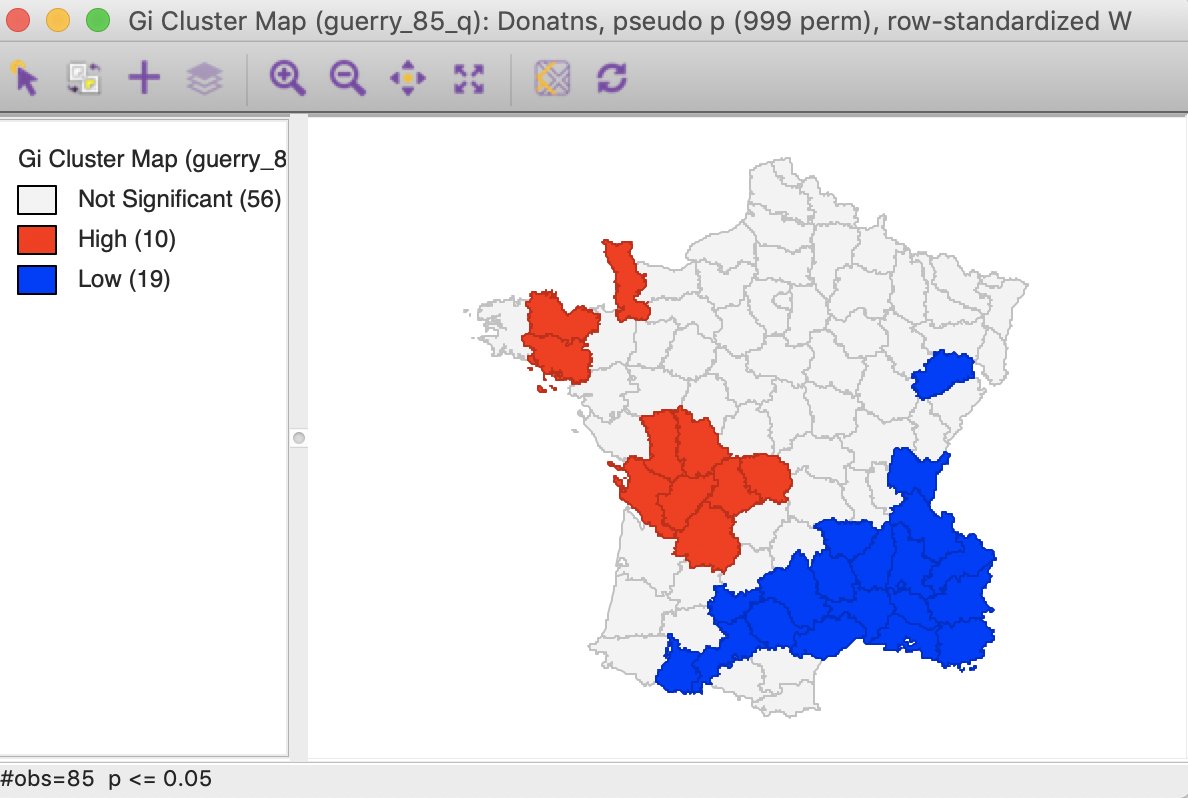Figure 46: Gi statistic default cluster map (999 permutations)

In this particular example, the cluster map for the $$G_i^*$$ statistic, shown in Figure 47, gives the identical results. This is often the case, but not always, so there is a point in computing both statistics.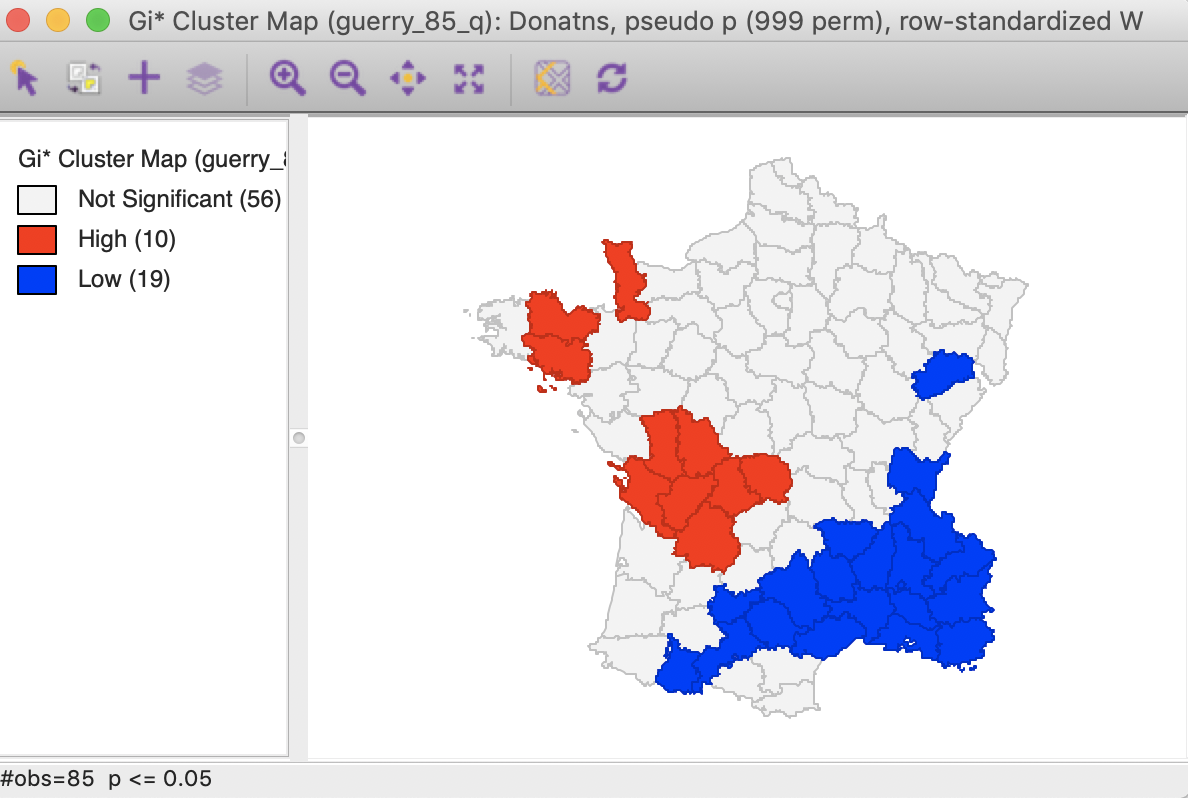Figure 47: Gi* statistic default cluster map (999 permutations)

For the Getis-Ord statistics, all the same options are available as for the Local Moran and the Local Geary statistics, and we refer to those discussions for details.

### Interpretation and significance

In the same way as for the other statistics, changing the number of permutations to 99999 provides a more detailed insight into the importance of the different locations, as indicated in the significance map. While 21 locations are deemed to be significant for 0.05, there are only four such locations for 0.01, three for 0.001 and one for 0.00001. This is the exact same result as for the Local Moran, illustrated in Figure 48 for the $$G_i$$ statistic (the results for the $$G_i^*$$ statistic are the same).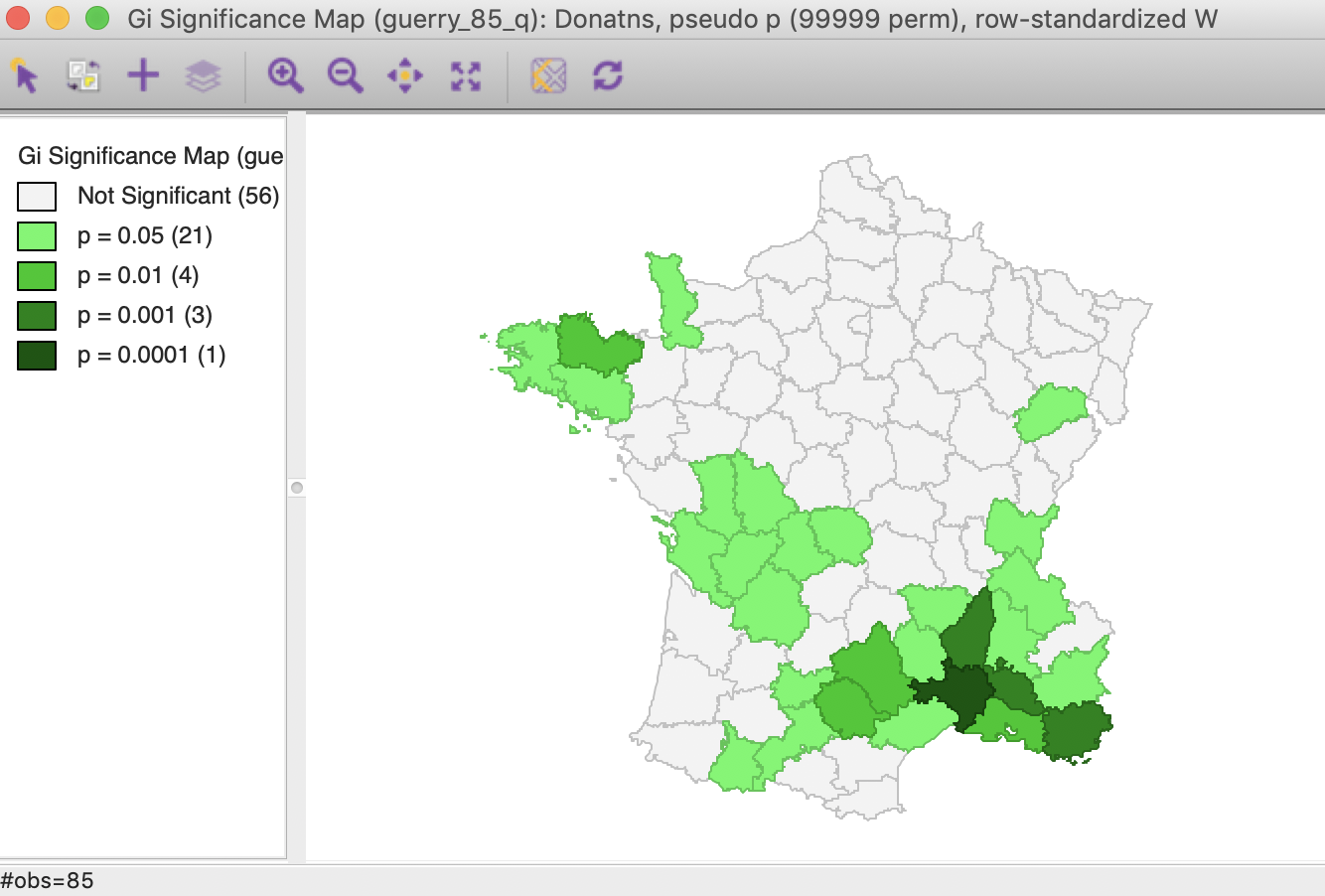Figure 48: Gi statistic significance map (99999 permutations)

Using the Significance Filter, we can assess the effect of a change of critical p-value to 0.01. In Figure 49, only one high-high cluster core remains, whereas the low-low cluster is reduced to seven observations.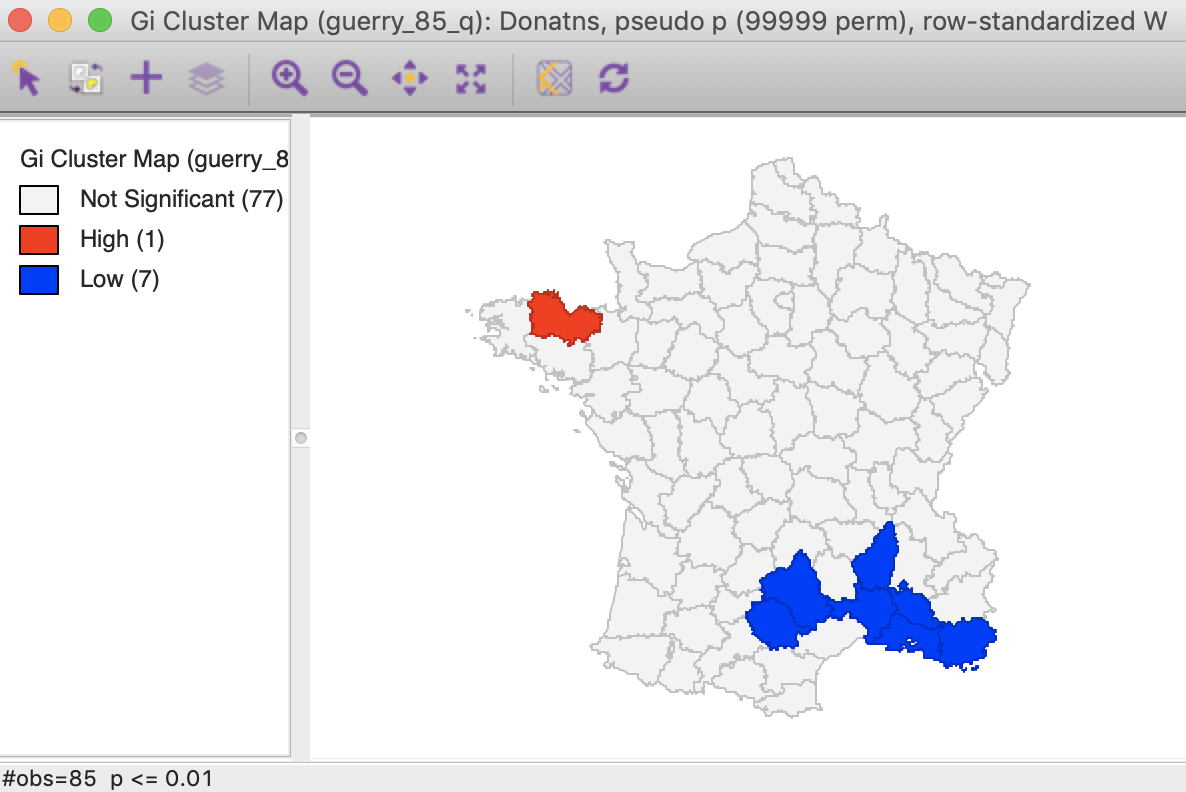Figure 49: Gi statistic cluster map (p < 0.01)

As shown in Figure 50, the FDR criterion further reduces the number of significant locations to three in the South of the country. These are the same three locations also identified by the Local Moran statistic.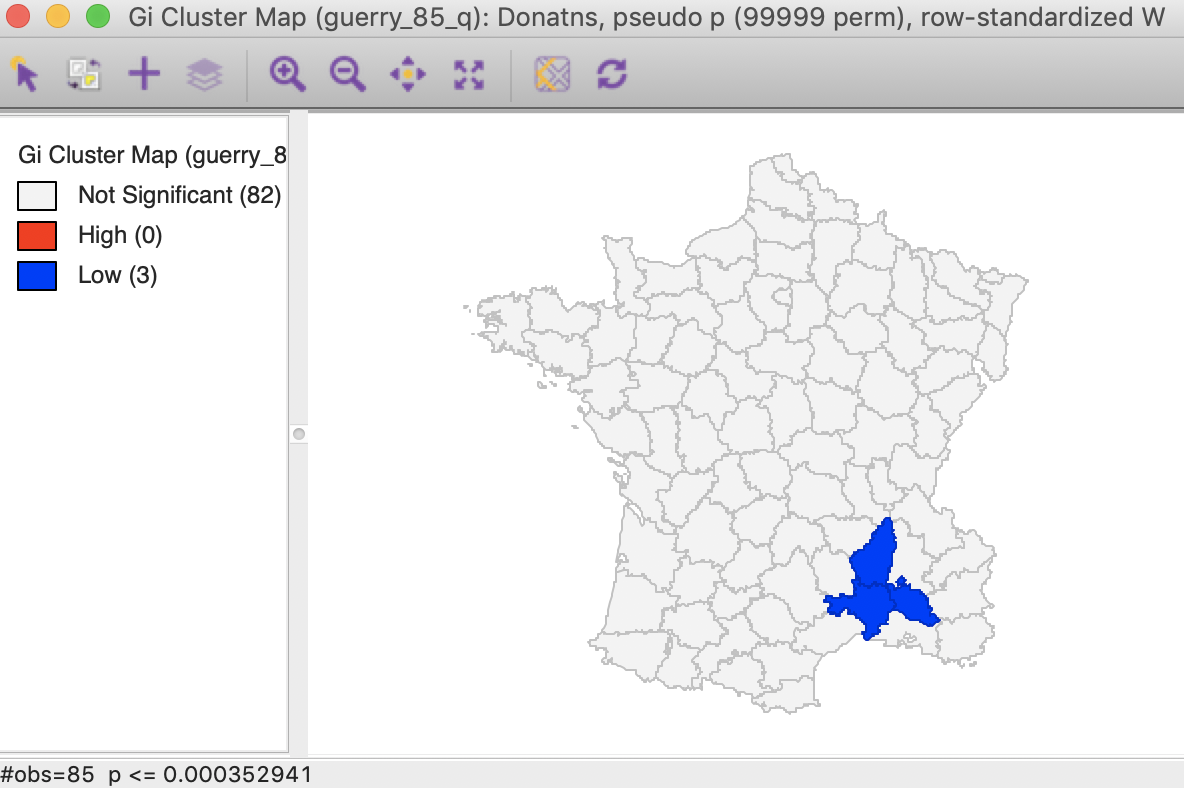Figure 50: Gi statistic cluster map (FDR)

The result for the Bonferroni bound is again the same as for the Local Moran, with only one significant location (see Figure 23).

#### Saving the results

The Save Results option makes it possible to add the statistics and their characteristics to the data table. As shown in Figure 51, three options are available: the statistic itself (either $$G_i$$ or $$G_i^*$$), the associated cluster category and pseudo p-values.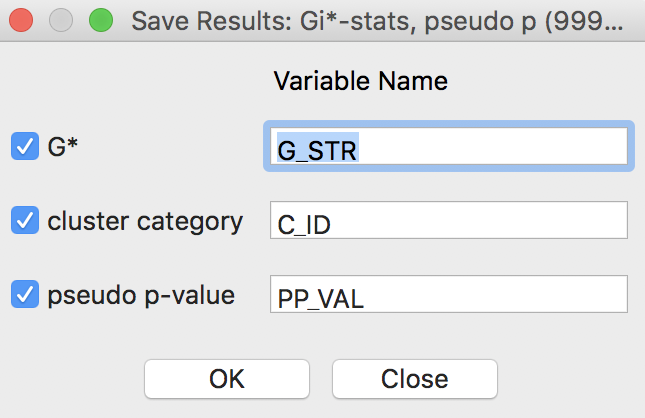Figure 51: Getis-Ord statistics Save Results options

For the Getis-Ord statistics, there are only three cluster categories, with observations taking the value of 0 for not significant, 1 for a high-high cluster, and 2 for a low-low cluster.

As always, the addition of the new variables to the table is made permanent by a save operation.

## Local Join Count Statistic

### Principle

Recently, Anselin and Li (2019) showed how a constrained version of the $$G_i^*$$ statistic yields a local version of the well-known join count statistic for spatial autocorrelation of binary variables, popularized by Cliff and Ord (1973). Expressed as a LISA statistic, a local version of the so-called BB join count statistic is $BB_i = x_i \sum_j w_{ij}x_j,$ where $$x_{i,j}$$ can only take on the values of 1 and 0, and $$w_{ij}$$ are the elements of a binary spatial weights matrix (i.e., not row-standardized). For the most meaningful results, the value of 1 should be chosen for the case with the fewest observations (of course, the definition of what is 1 and 0 can easily be switched).

The statistic is only meaningful for those observations where $$x_i = 1$$, since for $$x_i = 0$$ the result will always equal zero. A pseudo p-value is obtained by means of a conditional permutation approach, in the same way as for the other local spatial autocorrelation statistics, but only for those observations with $$x_i = 1$$. The same caveats as before should be kept in mind when interpreting the results, which are subject to multiple comparisons and the sensitivity of the pseudo p-value to the actual simulation experiment (random seed, number of permutations). Technical details are provided in Anselin and Li (2019).

### Implementation

#### Preliminaries

To illustrate the implementation of the Local Join Count (or local BB) statistic, we will use a somewhat contrived example. The main point is to show how the statistic works and how it can be interpreted, not any substantive concern.4

We continue with the variable Donatns and will turn the continuous distribution reflected in the natural breaks map in Figure 3 into a binary variable (the map is based on 6 categories). We select the three top categories, which yields 16 observations, shown in Figure 52.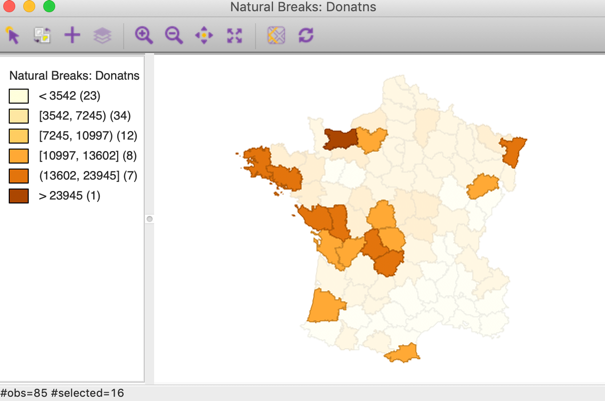Figure 52: Selected observations

We next use the Save Selected option in the Table to create a binary variable that corresponds to the selection (for the sake of the example, we use the default variable name of SELECTED). The resulting unique values map (with the color for the 0 category set to white) is as in Figure 53.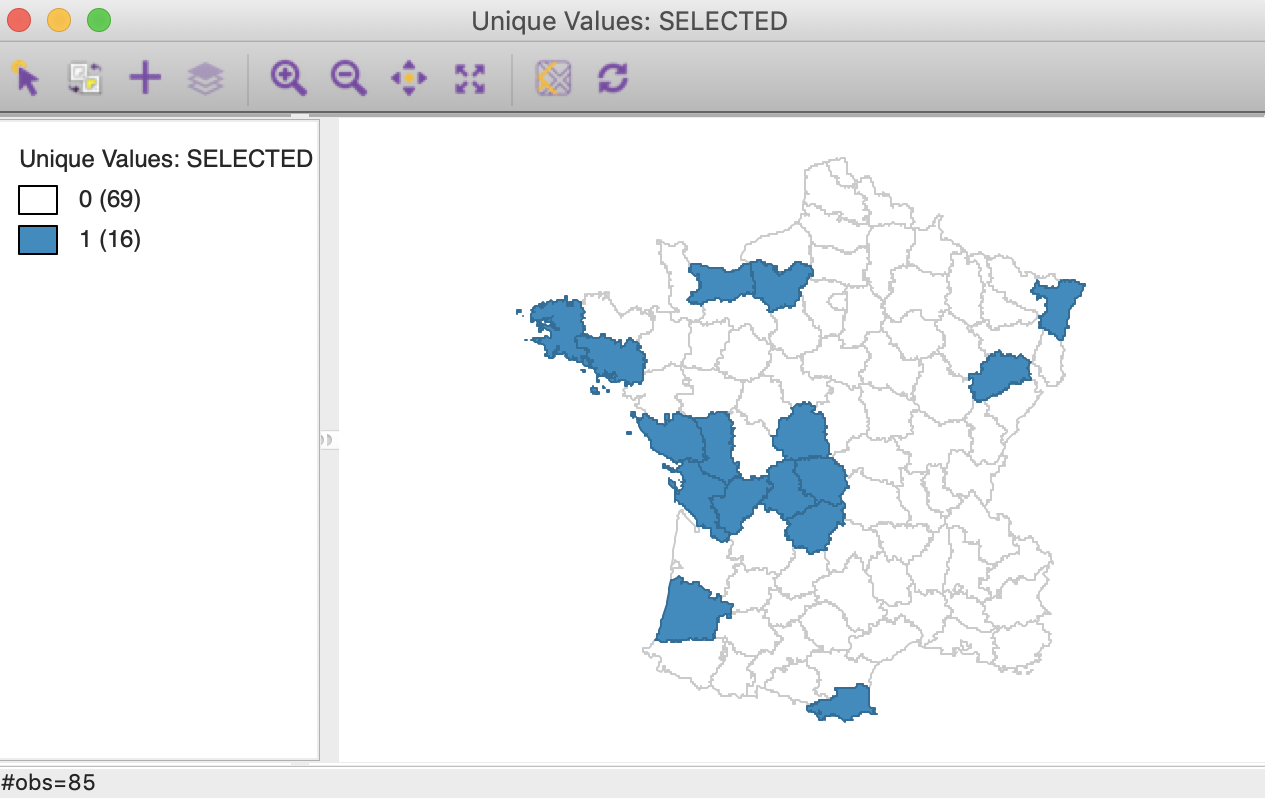Figure 53: Selected observations

A cursory inspection of the map reveals several singletons and pairs, with a larger grouping of like observations near the center of the map. The local join count statistic comes into play to assess the extent to which this perception of a cluster has any degree of significance (in the limited sense used here).

#### Cluster map

The statistic is invoked from the cluster map toolbar icon as Univariate Local Join Count, as shown in Figure 54. Alternatively, one can use Space > Univariate Local Join Count from the menu.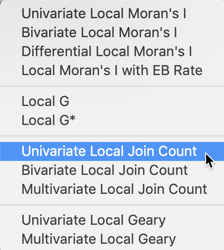Figure 54: Univariate Local Join Count from cluster maps toolbar options

Next, we use SELECTED from the Variable Settings dialog. In contrast to the other univariate local spatial autocorrelation statistics, there is no cluster map, only a significance map. Hence there is no need for a dialog to choose which windows are requested.

With the default significance level of 0.05 and 999 permutations, the result appears as in Figure 55.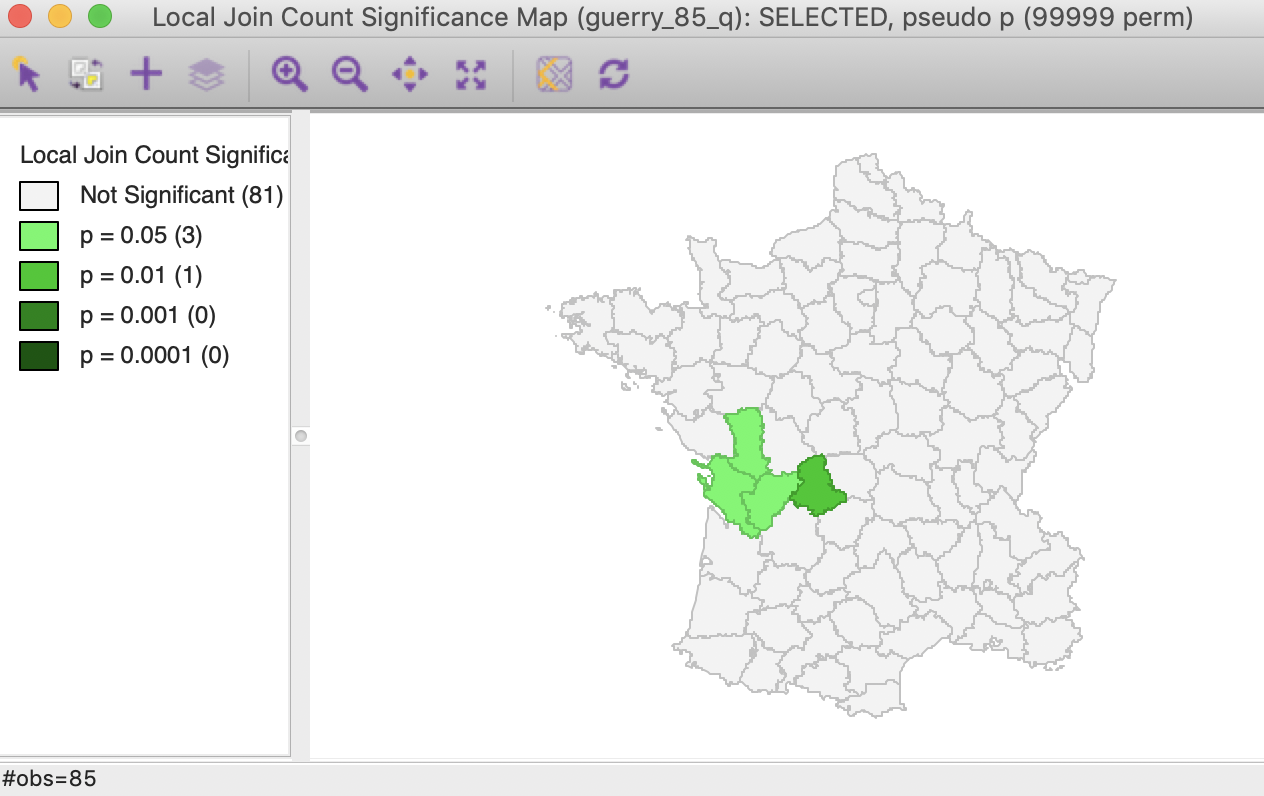Figure 55: Local Join Count Significance Map

Only four locations are deemed to be significant, three at 0.05 and one at 0.01. The minimum level p-value of 0.001 (with 999 permutations) is not reached for any of these observations.

All the usual options for local statistics apply here as well, i.e., changing the randomization option, the significance filter, and selecting cores and neighbors. To illustrate the latter, we select the observation with p = 0.01 as the core, and choose Select All > Cores and Neighbors. This highlights the observation in question and its (queen contiguity) neighbors in all other maps, including the unique value map from Figure 53. The result is the cluster shown in Figure 56, with four of the six neighbors of the observation in question also taking the value of 1. The other significant locations have three out of five neighbors with the value of 1, which turns out to be less significant.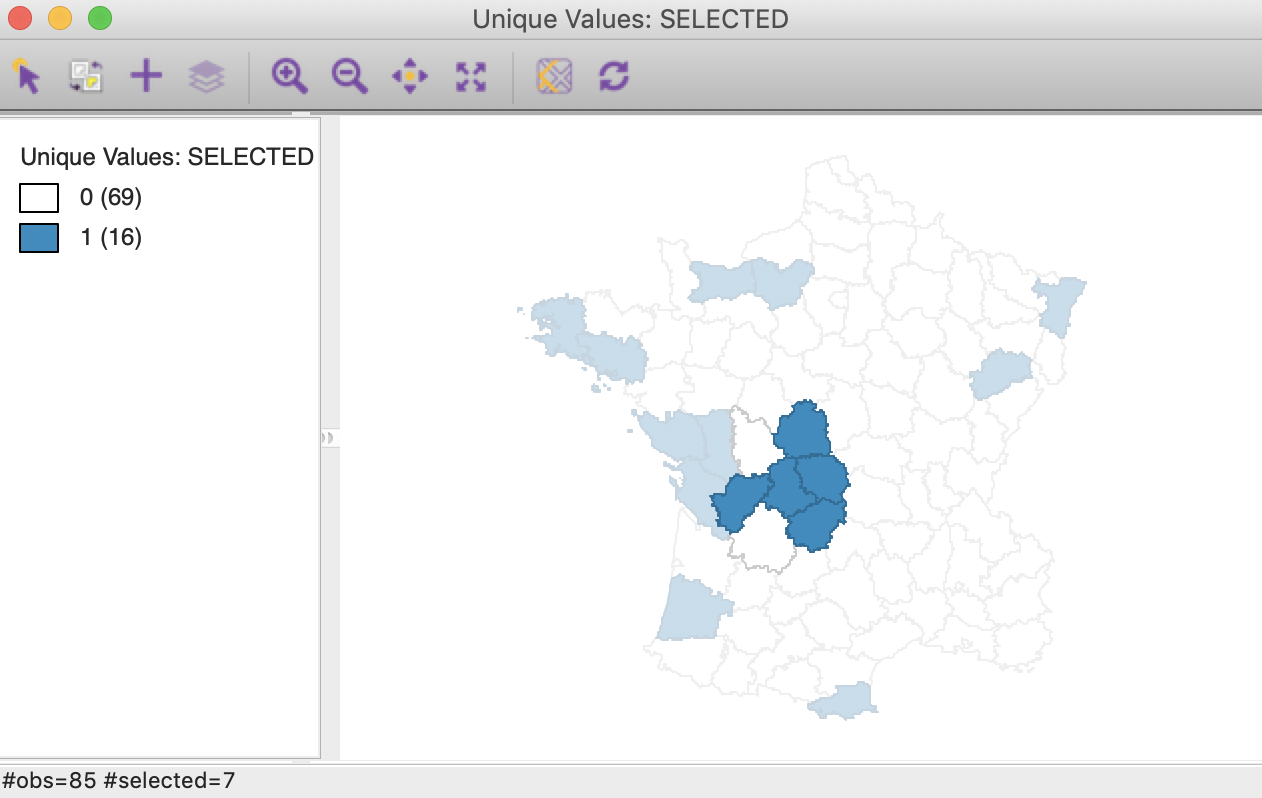Figure 56: Local Join Count Cluster Core with Neighbors

#### Saving the results

An alternative way to check on the neighbor structure of the significant locations is to use the Save Results option. Similar to its operation for the other local spatial autocorrelation statistics, this saves the statistic, i.e., the number of BB joins (default variable name JC), the total number of neighbors (NN), and the pseudo p-value (PP_VAL), as in Figure 57. As usual, the new variables are only permanently added to the Table after a Save command.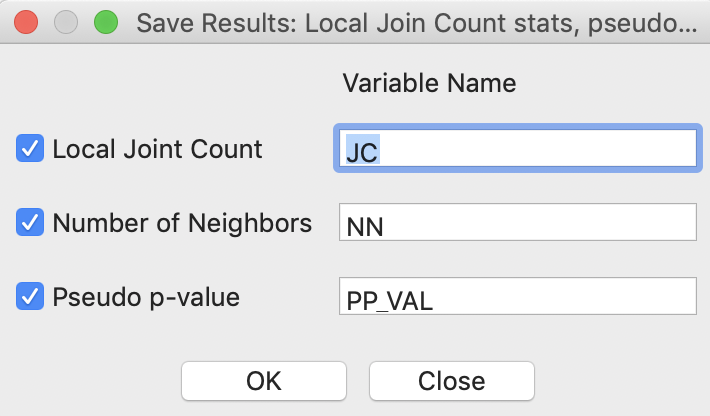Figure 57: Local Join Count Save Results options

Selecting the four significant locations and moving them to the top of the table, shows the corresponding values, as in Figure 58. This matches what our visual inspection revealed. In larger data sets, it may be more difficult to carry out a visual inspection, and the table entries may be more insightful.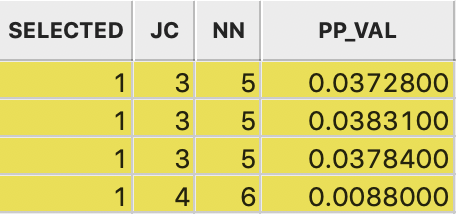Figure 58: Local Join Count Results in Table

1. University of Chicago, Center for Spatial Data Science –

2. Again, we accomplish this in the Table Calculator. First, we create a new column/variable as FDR, with the constant value $$\alpha / n$$, using Univariate > Assign. Next, we multiply the FDR value with the value for I, using Bivariate > Multiply.

3. When all observations for a variable are positive, as is the case in our examples, the G statistics are positive ratios less than one. Large ratios (more precisely, less small values since all ratios are small) correspond with high-high hot spots, small ratios with low-low cold spots.

4. For a more realistic example, see the empirical illustration in Anselin and Li (2019).